# Exploratory Factor analysis

### Libraries used:

• psych
• GPArotation
• factoextra

PCA and SVD are considered simple forms of exploratory factor analysis. The term ‘factor analysis’ is a bit confusing and you will find a variety of definitions out there–some people assert that PCA is not factor analysis, and others might use PCA but call it factor analysis. PCA involves a complete redescription of the covariance or correlation matrix along a set of independent dimensions, until it completely redescribes the data, and then you pick off the most important components and ignore the rest. EFA is typically attempting to do the same thing, but trying to maximize the variance accounted for by a fixed number of ‘latent’ factors, so that we have the model + error. Furthermore, most of the time factor analysis lets us pick orthogonal dimensions based on criteria other than maximum variance, and permits factors that are not orthogonal, allowing for latent factors that are somewhat related.

Making an analogy to MDS, classic MDS used eigen decomposition and then peels off just the top dimensions. But sammon and non-metric scaling try to force the higher dimensions into fewer. Now, instead of fitting all the noise, we can minimize or optimize some notion of fitting the correlation matrix, and treat everything else as noise. When an algorithm has this goal, it is typically called ‘factor analysis’. As such, it is important when you report an analysis to give specific details of how the analysis was performed, including the software routine and arguments used.

# A simple example

In the section on PCA, we examined human abilities and came up with a solution in which one component was an overall ability across all skills, one was visual vs. verbal, and a third was picture vs. maze:

library(GPArotation)
data(ability.cov)
e2 <- eigen(cov2cor(ability.cov$cov)) plot(e2$values)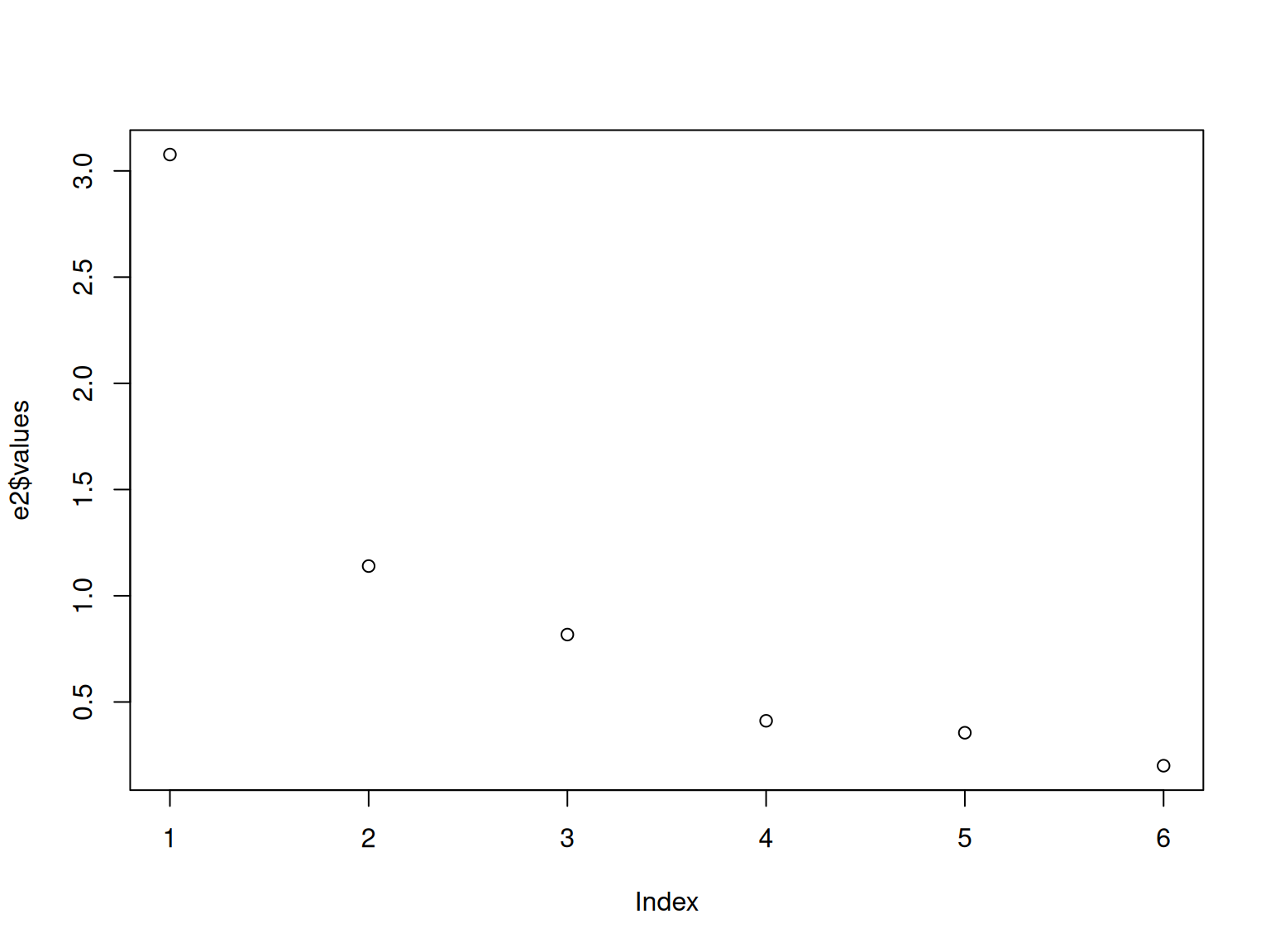evec <- e2$vectors rownames(evec) <- rownames(ability.cov$cov)
round(evec, 3)
          [,1]   [,2]   [,3]   [,4]   [,5]   [,6]
general -0.471  0.002  0.072  0.863  0.037 -0.164
picture -0.358  0.408  0.593 -0.268  0.531  0.002
blocks  -0.434  0.404  0.064 -0.201 -0.775  0.051
maze    -0.288  0.404 -0.794 -0.097  0.334  0.052
reading -0.440 -0.507 -0.015 -0.100  0.056  0.733
vocab   -0.430 -0.501 -0.090 -0.352  0.021 -0.657
barplot(t(evec[, 1:3]), beside = T)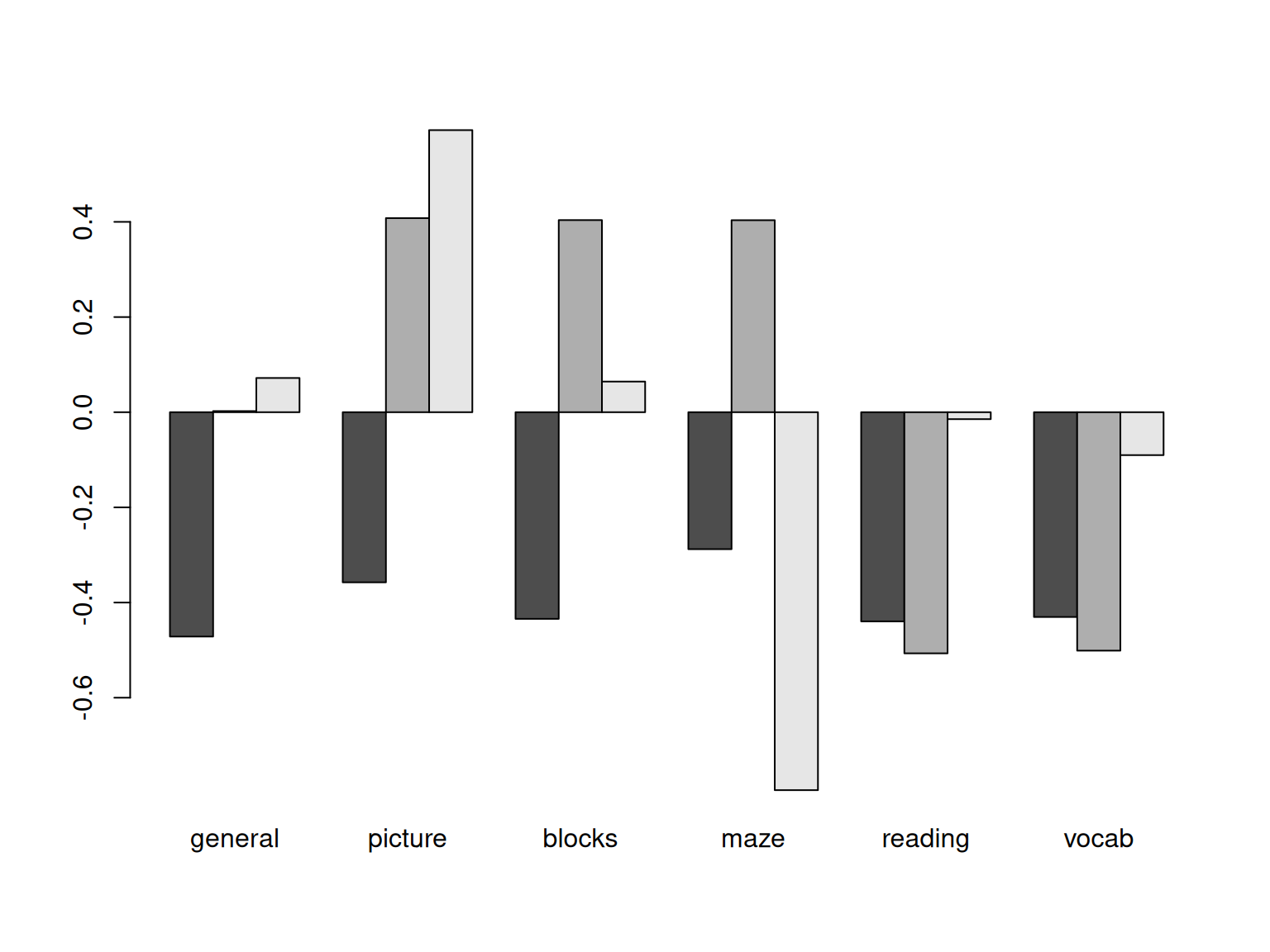We can use the factanal function to do something similar, giving it the covariance matrix. We now specify exactly how many factors we want:

f3 <- factanal(covmat = ability.cov, factors = 3)
print(f3)

Call:
factanal(factors = 3, covmat = ability.cov)

Uniquenesses:
general picture  blocks    maze reading   vocab
0.441   0.217   0.329   0.580   0.040   0.336

Factor1 Factor2 Factor3
general 0.499   0.394   0.393
picture 0.133   0.862   0.153
blocks  0.230   0.525   0.586
maze    0.110           0.632
vocab   0.782   0.123   0.192

Factor1 Factor2 Factor3
Proportion Var   0.312   0.203   0.161
Cumulative Var   0.312   0.515   0.676

The degrees of freedom for the model is 0 and the fit was 0 
barplot(t(loadings(f3)), beside = T)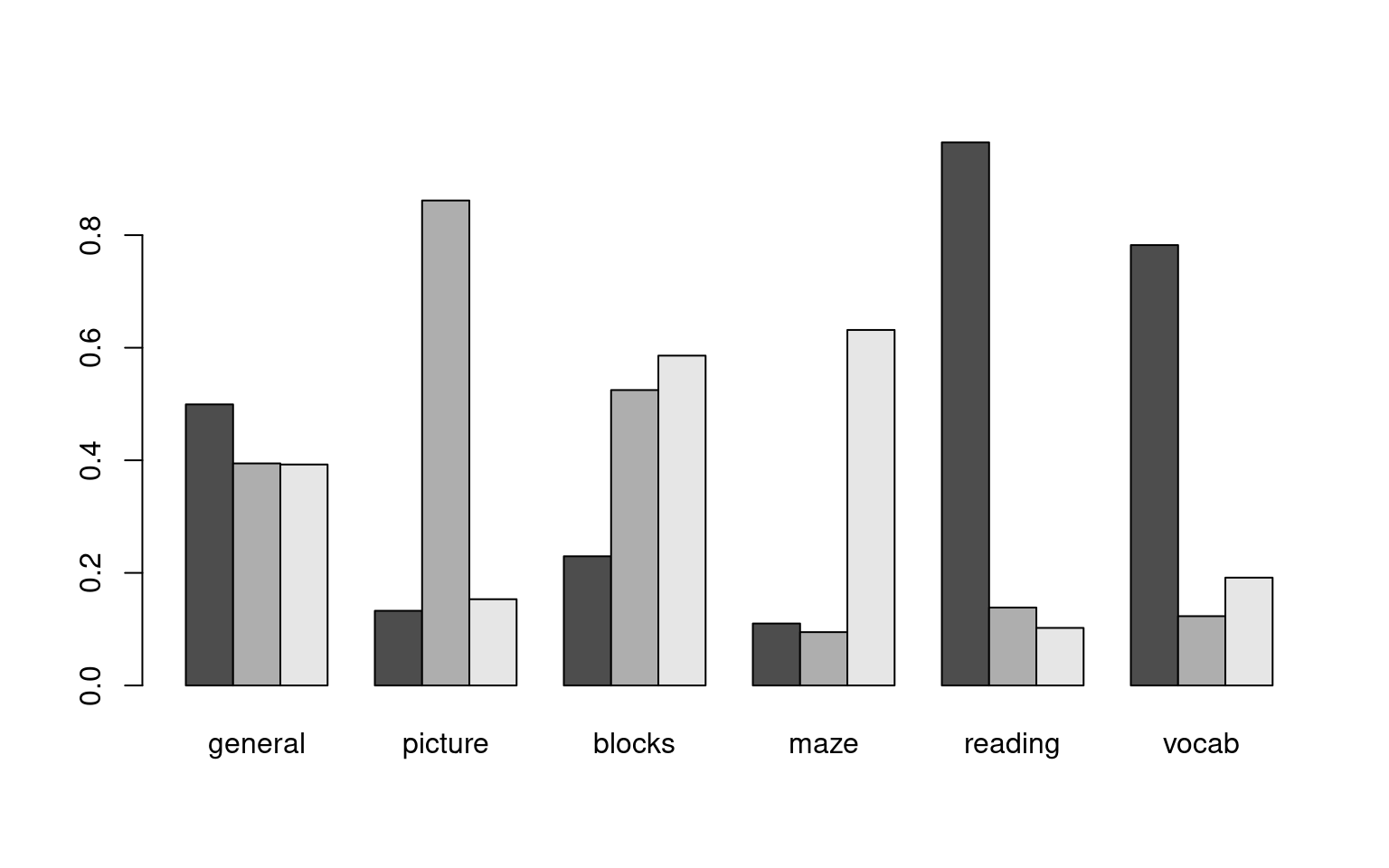Now, the first three factors turn out a bit differently. factor1 is the specific general skill, reading and vocab–a basic verbal ability. Factor 2 is picture+books; the visual skills that don’t involve maze, plus some general. The third loads highly on blocks, maze, and general. These tend to focus on single common positively-correlated factors rather than the divisive components we happened to get with PCA. If you think about it, a mixture of these three factors can produce basically the same patterns as the first three factors of the PCA, but in a different way. Note that the PCA dimensions do not change as we increase the number of dimensions–we do this once and pick off the first few dimensions we care about. FA gives us the best model with a limited number of factors (usually from a maximum likelihood perspective), and it calculates a statistical test asking whether the model is sufficient, at least if it can compute this For f3, it cannot be calculated, and it says the DF of the model is 0 and the fit is 0. For 1- and 2-factor models, it tells us that 1-factor fails but 2-factor does not, suggesting a 2-factor solution is sufficient.

f1 <- factanal(covmat = ability.cov, factors = 1)
f2 <- factanal(covmat = ability.cov, factors = 2)

f1

Call:
factanal(factors = 1, covmat = ability.cov)

Uniquenesses:
general picture  blocks    maze reading   vocab
0.535   0.853   0.748   0.910   0.232   0.280

Factor1
general 0.682
picture 0.384
blocks  0.502
maze    0.300
vocab   0.849

Factor1
Proportion Var   0.407

Test of the hypothesis that 1 factor is sufficient.
The chi square statistic is 75.18 on 9 degrees of freedom.
The p-value is 1.46e-12 
f2

Call:
factanal(factors = 2, covmat = ability.cov)

Uniquenesses:
general picture  blocks    maze reading   vocab
0.455   0.589   0.218   0.769   0.052   0.334

Factor1 Factor2
general 0.499   0.543
picture 0.156   0.622
blocks  0.206   0.860
maze    0.109   0.468
vocab   0.785   0.225

Factor1 Factor2
Proportion Var   0.310   0.287
Cumulative Var   0.310   0.597

Test of the hypothesis that 2 factors are sufficient.
The chi square statistic is 6.11 on 4 degrees of freedom.
The p-value is 0.191 

Note that each variable is given a ‘uniqueness’ score, and we can see that the measures that were associated with others have lower scores. This can help identify whether there are measures that should be removed, because they are simply multiple measures of the same thing. Also, the factors show sum-of-squares (variace), proportion and cumulative, to give a basic effect size for each factor. Because the factors try to maximize variance, we see less of a fall-off in explained variance across factors, and the met hod balances variance more equally across factors. However, as we increase N, you can see the total additional variance accounted for gets smaller. In this case, f1 accounted for 41%, f2 accounted for 50%, and f3 accounted for 67%.

Now that we see some of the similarities and differences, let’s examine the different arguments to factor analysis.

## Optimization method.

Factor analysis can be thought of as a model of the correlation matrix of the responses that uses a fixed number of predictors to describe the data. How this works is that the factor analysis method essentially tries to do PCA using an iterative approximation, with the restriction that you are forced to use a fixed number of factors. After starting at some solution, it iteratively refines this to best describe the data better and maximize goodnees of fit of the model or minimize error in order to best describe the covariance data. However, it is not always exactly clear what ‘best describe the data’ means. Some traditional methods are ‘minres’, maximum likelihood, and principal axes, but other methods are available for special cases, depending on the software. In general, choice of optimization method probably depends more on requirements such as size, missing values, availability of within software etc.–the results might not differ much, one probably won’t be universally better for all data sets, and results will be more impacted by other things such as the rotation. The literature suggests that the particular optimization methods differ across software packages, are not well documented, and typically produce similar results. Most sources appear to recommend using ML as a default if the matrix is well-behaved, and if it is not (i.e., the model does not converge) to try another method. The ML (maximum likelihood) factor analysis attempts to find a solution that is most likely to have produced the correlation matrix. Some methods will warn about ‘Heywood cases’ which could signal that another optimization method could be tried.

The factanal function in R uses ML and has a number of options for controlling the estimation; the fa function in in the psych offers more alternatives to ML, but its ML implementation has fewer options. We will also use the psych::fa function because of better output, but you might try an alternate implementation if you run into trouble.

## Rotations

Once a solution has been produced, we will have a small fixed number of components/factors that hopefully do a good job a redescribing the complete correlation matrix. But we have a potential problem of non-identifiability. These base factors will be orthogonal just as in PCA, which means that any point (i.e, a response pattern across questions) can be described as a combination of factors. But there are rotations of these axes that produce equally good fits. Thus, once the analysis is done, one usually chooses one of a number of rotations for looking at the data. Some common ones are available within the GPArotation library in R, and can be applied either as a function of fa, or afterward.

It might seem non-intuitive that the rotation is not a part of the optimizization. But unlike PCA which has a very well-defined way of assigning dimensions (each dimension maximizes variance in that direction, subject to being orthogonal to all others), factor analysis finds its solution to minimize residual variance/maximize goodness-of-fit. Consequently, it is more like MDS, in that a particular dimension that it picks may not be the best dimension to look at. A specific rotation may help, and often leads to more interpretable factors, because they are designed to hang together better and account for distinct variance. These rotations are available within several packages, including GPArotation, which is used by psych::fa.

• varimax (variance maximizing rotation). This maximizes the variance of the factors. Because variance involves squared deviation, it is best to try to put all the variance of any question into a single factor, rather than spreading it out. So overall, factors should favor all-or-none membership of items. Thus it tries to align factors with measures that vary. This is the most popular orthogonal rotation method used frequently by default, and aims to find a simple model where questions are related to unique factors. Within psych::fa, it is no longer the default; as that package uses a non-orthogonal rotation.

• quartimax. This maximizes the variance in each item (rather than each factor). Again, this aims for a simple model. To maximize the variance of each item, it should also be placed in a single factor, so it is described as minimizing the number of factors an item appears in.

• equamax uses a weighted sum of varimax and quartimax.

• varimin a does the opposite of what varimax does.

• A list of available orthogonal rotations includes: “none”, “varimax”, “quartimax”, “bentlerT”, “equamax”, “varimin”, “geominT” and “bifactor”

The above are all ‘orthogonal’ rotations–they require the factors to be independent. But it may be better if we allow the factors to be somewhat correlated if they are able to simplify the model more. These are not exactly rotations, because they are also essentially warping the dimensions of the space. They are more properly called ‘oblique transformations’.

The oblique transformations are harder to easily describe. They use differing criteria to try to create simple and interpretable models. For oblique transformations, the factors will be correlated with one another. However, the correlation cannot be too large, because then it would be better to combine those factors into a single factor.

Some options include the following. These are not well documented, and so it is usually preferable to use one that is well understood than to use one you cannot justify, but sometimes an exotic rotation will show a view of your data you did not see anywhere else.

• oblimin (default of the fa function).
• promax: an oblique transformation that has been decribed as having similar goals to varimax.
• simplimax
• bentlerQ
• geominQ
• quartimin
• biQuartmin
• cluster

# Example: Big Five data set

Let’s try reading in the big-five data set and performing a factor analysis with various different rotations. We will use the big five data, and both fa and factanal will take the entire data set directly, and not require you to compute a correlation matrix first.

## Read in the big-five data set:

data <- read.csv("bigfive.csv")

dat.vals <- data[, -1]  ##remove subject code
qtype <- c("E", "A", "C", "N", "O", "E", "A", "C", "N", "O", "E", "A", "C",
"N", "O", "E", "A", "C", "N", "O", "E", "A", "C", "N", "O", "E", "A", "C",
"N", "O", "E", "A", "C", "N", "O", "E", "A", "C", "N", "O", "O", "A", "C",
"O")
valence <- c(1, -1, 1, 1, 1, -1, 1, -1, -1, 1, 1, -1, 1, 1, 1, 1, 1, -1, 1,
1, -1, 1, -1, -1, 1, 1, -1, 1, 1, 1, -1, 1, 1, -1, -1, 1, -1, 1, 1, 1, -1,
1, -1, 1)
add <- c(6, 0, 0)[valence + 2]

tmp <- dat.vals
tmp[is.na(tmp)] <- 3.5
reversed <- t(t(tmp) * valence + add)
## reverse code questions:

bytype <- reversed[, order(qtype)]
key <- sort(qtype)
colnames(bytype) <- paste(key, 1:44, sep = "")

##Factor analysis functions

First, let’s confirm that the different fa methods can produce similar results:

## factanal is built-in to R
f1a <- factanal(bytype, factors = 3)
library(psych)
f1b <- fa(bytype, nfactors = 3, rotate = "varimax")
f1a

Call:
factanal(x = bytype, factors = 3)

Uniquenesses:
A1    A2    A3    A4    A5    A6    A7    A8    A9   C10   C11   C12
0.859 0.772 0.844 0.883 0.912 0.896 0.748 0.840 0.829 0.766 0.833 0.768
C13   C14   C15   C16   C17   C18   E19   E20   E21   E22   E23   E24
0.836 0.820 0.770 0.797 0.815 0.835 0.450 0.521 0.589 0.502 0.447 0.734
E25   E26   N27   N28   N29   N30   N31   N32   N33   N34   O35   O36
0.599 0.543 0.633 0.661 0.663 0.614 0.656 0.727 0.799 0.707 0.674 0.691
O37   O38   O39   O40   O41   O42   O43   O44
0.753 0.667 0.728 0.738 0.984 0.685 0.912 0.797

Factor1 Factor2 Factor3
A1   0.367
A2   0.276   0.386
A3   0.328   0.125  -0.181
A4   0.250   0.233
A5   0.199   0.200
A6   0.278   0.105   0.125
A7   0.231   0.439
A8   0.354   0.160
A9   0.206   0.333   0.132
C10  0.277   0.397
C11  0.405
C12  0.278   0.393
C13  0.401
C14  0.398           0.121
C15  0.242   0.409
C16  0.299   0.318   0.111
C17  0.250   0.343
C18  0.403
E19          0.156   0.721
E20                  0.682
E21  0.205   0.287   0.535
E22  0.132   0.335   0.607
E23         -0.111   0.735
E24                  0.512
E25  0.236           0.585
[ reached getOption("max.print") -- omitted 19 rows ]

Factor1 Factor2 Factor3
Proportion Var   0.096   0.088   0.083
Cumulative Var   0.096   0.183   0.266

Test of the hypothesis that 3 factors are sufficient.
The chi square statistic is 5675.51 on 817 degrees of freedom.
The p-value is 0 
f1b
Factor Analysis using method =  minres
Call: fa(r = bytype, nfactors = 3, rotate = "varimax")
MR1   MR2   MR3    h2   u2 com
A1   0.39  0.03  0.04 0.156 0.84 1.0
A2   0.33  0.38  0.02 0.250 0.75 2.0
A3   0.37  0.12 -0.22 0.197 0.80 1.9
A4   0.27  0.22  0.01 0.123 0.88 1.9
A5   0.23  0.18  0.07 0.091 0.91 2.2
A6   0.33  0.11  0.07 0.123 0.88 1.3
A7   0.29  0.44 -0.12 0.293 0.71 1.9
A8   0.40  0.16 -0.14 0.201 0.80 1.6
A9   0.25  0.33  0.10 0.178 0.82 2.1
C10  0.32  0.37  0.01 0.244 0.76 2.0
C11  0.44  0.00 -0.06 0.198 0.80 1.0
C12  0.34  0.37 -0.05 0.255 0.75 2.0
[ reached getOption("max.print") -- omitted 32 rows ]

MR1  MR2  MR3
Proportion Var        0.10 0.09 0.08
Cumulative Var        0.10 0.18 0.27
Proportion Explained  0.37 0.32 0.31
Cumulative Proportion 0.37 0.69 1.00

Mean item complexity =  1.5
Test of the hypothesis that 3 factors are sufficient.

The degrees of freedom for the null model are  946  and the objective function was  14.28 with Chi Square of  14284.11
The degrees of freedom for the model are 817  and the objective function was  5.72

The root mean square of the residuals (RMSR) is  0.07
The df corrected root mean square of the residuals is  0.08

The harmonic number of observations is  1017 with the empirical chi square  9890.49  with prob <  0
The total number of observations was  1017  with Likelihood Chi Square =  5714.55  with prob <  0

Tucker Lewis Index of factoring reliability =  0.574
RMSEA index =  0.078  and the 90 % confidence intervals are  0.075 0.079
BIC =  57.14
Fit based upon off diagonal values = 0.83
MR1  MR2  MR3
Correlation of (regression) scores with factors   0.92 0.91 0.92
Multiple R square of scores with factors          0.84 0.83 0.85
Minimum correlation of possible factor scores     0.68 0.66 0.70

Notice that the two methods produce identical results, but they display different information and show the factors differently. Let’s focus on the output of fa, which was done using the minres method; we could have also selected ml, wls, gls, or pa.

• It first shows a matrix that includes the three factors (MR2, MR3, and MR1), along with several other statistics. You can get this by doing loadings(model). This will display only the ‘important’ values–the values close to 0 will not be shown for easier examination.
• h2 is the communality score–sum of the squared factor loadings for each question. Somewhat like an $$R^2$$ value for each item.
• u2 is the uniqueness score, 1-h2.
• com is complexity, an information score that is generally related to uniqueness.

Next, a number of statistics related to the model are shown. It then provides a chi-squared test of whether the model is sufficiently complex (or needs more factors), and other diagnostics of whether the model is acceptable. Because we guess there might be five, we will try five, using the ml optimization method

## Optimization method

let’s look at different optimization methods, using the fm argument

f2 <- psych::fa(bytype, nfactors = 5, fm = "ml", rotate = "quartimax")
f2
Factor Analysis using method =  ml
Call: psych::fa(r = bytype, nfactors = 5, rotate = "quartimax", fm = "ml")
ML2   ML3   ML4   ML1   ML5   h2   u2 com
A1   0.10 -0.27 -0.05  0.08  0.33 0.20 0.80 2.3
A2   0.11 -0.07  0.17  0.34  0.35 0.29 0.71 2.7
A3  -0.13 -0.16 -0.04  0.15  0.43 0.25 0.75 1.8
A4   0.06 -0.13  0.11  0.17  0.29 0.14 0.86 2.5
A5   0.12 -0.06  0.05  0.26  0.17 0.11 0.89 2.5
A6   0.20 -0.09 -0.09  0.12  0.49 0.31 0.69 1.6
A7   0.00  0.02  0.18  0.28  0.57 0.43 0.57 1.7
A8  -0.04 -0.19  0.01  0.05  0.62 0.42 0.58 1.2
A9   0.19 -0.01  0.12  0.26  0.37 0.26 0.74 2.6
[ reached getOption("max.print") -- omitted 35 rows ]

ML2  ML3  ML4  ML1  ML5
Proportion Var        0.08 0.08 0.07 0.07 0.05
Cumulative Var        0.08 0.16 0.23 0.30 0.35
Proportion Explained  0.23 0.22 0.21 0.19 0.16
Cumulative Proportion 0.23 0.45 0.66 0.84 1.00

Mean item complexity =  1.8
Test of the hypothesis that 5 factors are sufficient.

The degrees of freedom for the null model are  946  and the objective function was  14.28 with Chi Square of  14284.11
The degrees of freedom for the model are 736  and the objective function was  3.19

The root mean square of the residuals (RMSR) is  0.05
The df corrected root mean square of the residuals is  0.05

The harmonic number of observations is  1017 with the empirical chi square  4045.22  with prob <  0
The total number of observations was  1017  with Likelihood Chi Square =  3176.97  with prob <  3e-299

Tucker Lewis Index of factoring reliability =  0.764
RMSEA index =  0.058  and the 90 % confidence intervals are  0.055 0.059
BIC =  -1919.54
Fit based upon off diagonal values = 0.93
ML2  ML3  ML4  ML1  ML5
Correlation of (regression) scores with factors   0.93 0.92 0.91 0.88 0.88
Multiple R square of scores with factors          0.86 0.84 0.82 0.78 0.77
Minimum correlation of possible factor scores     0.73 0.68 0.65 0.56 0.53
f2b <- psych::fa(bytype, nfactors = 5, fm = "gls", rotate = "quartimax")
f2b
Factor Analysis using method =  gls
Call: psych::fa(r = bytype, nfactors = 5, rotate = "quartimax", fm = "gls")
GLS3  GLS4  GLS2  GLS1  GLS5   h2   u2 com
A1   0.11 -0.26 -0.08  0.07  0.38 0.23 0.77 2.1
A2   0.11 -0.06  0.19  0.30  0.39 0.30 0.70 2.6
A3  -0.14 -0.16 -0.04  0.14  0.45 0.27 0.73 1.7
A4   0.07 -0.12  0.11  0.11  0.36 0.18 0.82 1.7
A5   0.14 -0.05  0.05  0.22  0.23 0.13 0.87 2.9
A6   0.20 -0.09 -0.09  0.11  0.49 0.31 0.69 1.6
A7   0.00  0.02  0.22  0.22  0.58 0.44 0.56 1.6
A8  -0.05 -0.20  0.01  0.05  0.60 0.40 0.60 1.2
A9   0.20 -0.01  0.15  0.21  0.40 0.26 0.74 2.4
[ reached getOption("max.print") -- omitted 35 rows ]

GLS3 GLS4 GLS2 GLS1 GLS5
Proportion Var        0.08 0.08 0.07 0.06 0.06
Cumulative Var        0.08 0.16 0.23 0.30 0.35
Proportion Explained  0.23 0.22 0.21 0.18 0.16
Cumulative Proportion 0.23 0.45 0.66 0.84 1.00

Mean item complexity =  1.7
Test of the hypothesis that 5 factors are sufficient.

The degrees of freedom for the null model are  946  and the objective function was  14.28 with Chi Square of  14284.11
The degrees of freedom for the model are 736  and the objective function was  3.22

The root mean square of the residuals (RMSR) is  0.05
The df corrected root mean square of the residuals is  0.05

The harmonic number of observations is  1017 with the empirical chi square  4005.47  with prob <  0
The total number of observations was  1017  with Likelihood Chi Square =  3206.79  with prob <  3.1e-304

Tucker Lewis Index of factoring reliability =  0.761
RMSEA index =  0.058  and the 90 % confidence intervals are  0.055 0.06
BIC =  -1889.72
Fit based upon off diagonal values = 0.93
GLS3 GLS4 GLS2 GLS1 GLS5
Correlation of (regression) scores with factors   0.93 0.91 0.91 0.88 0.88
Multiple R square of scores with factors          0.86 0.83 0.83 0.78 0.77
Minimum correlation of possible factor scores     0.72 0.67 0.66 0.56 0.55
## causes trouble because of missing data:
pca <- princomp(bytype)

If you look at the loadings, values, etc. the factor analysis solutions are numerically different but tend to be fairly similar.

We can look at them specifically, especially in comparison to the PCA solution:

par(mfrow = c(3, 1))
matplot(loadings(f2), type = "s", lty = 1, main = "Factor analyis ML optimization")
matplot(loadings(f2b), type = "s", lty = 1, main = "Factor analysis GLS optimization")
matplot(loadings(pca)[, 1:5], main = "5 dimensions of PCA", type = "s", lty = 1)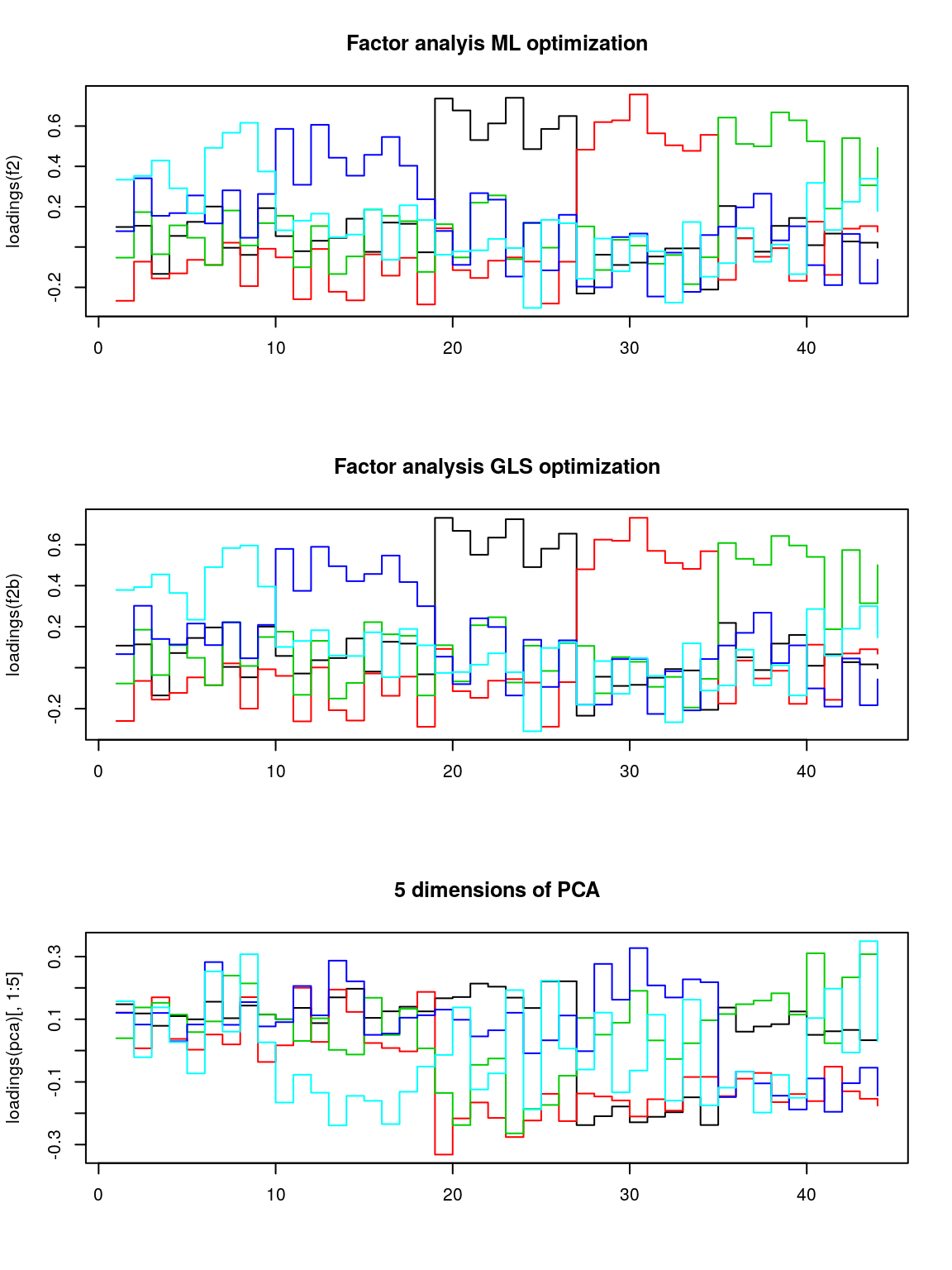That does not look bad. If you compare to the factor loadings from the PCA, these are much cleaner–the PCA is almost uninterpretable in comparison. Notice that the two optimization methods produce results that are essentially the same. It looks like each factor takes a turn and maps roughly onto a different set of personality questions. But maybe other rotations would provide a stronger factor structure. Although we applied rotations within the fa, we can also do it afterward:

library(GPArotation)  ##needed for rotations like oblimin
matplot(varimax(loadings(f2))$loadings, type = "s", lty = 1, main = "varimax rotation", ylab = "Factor loading")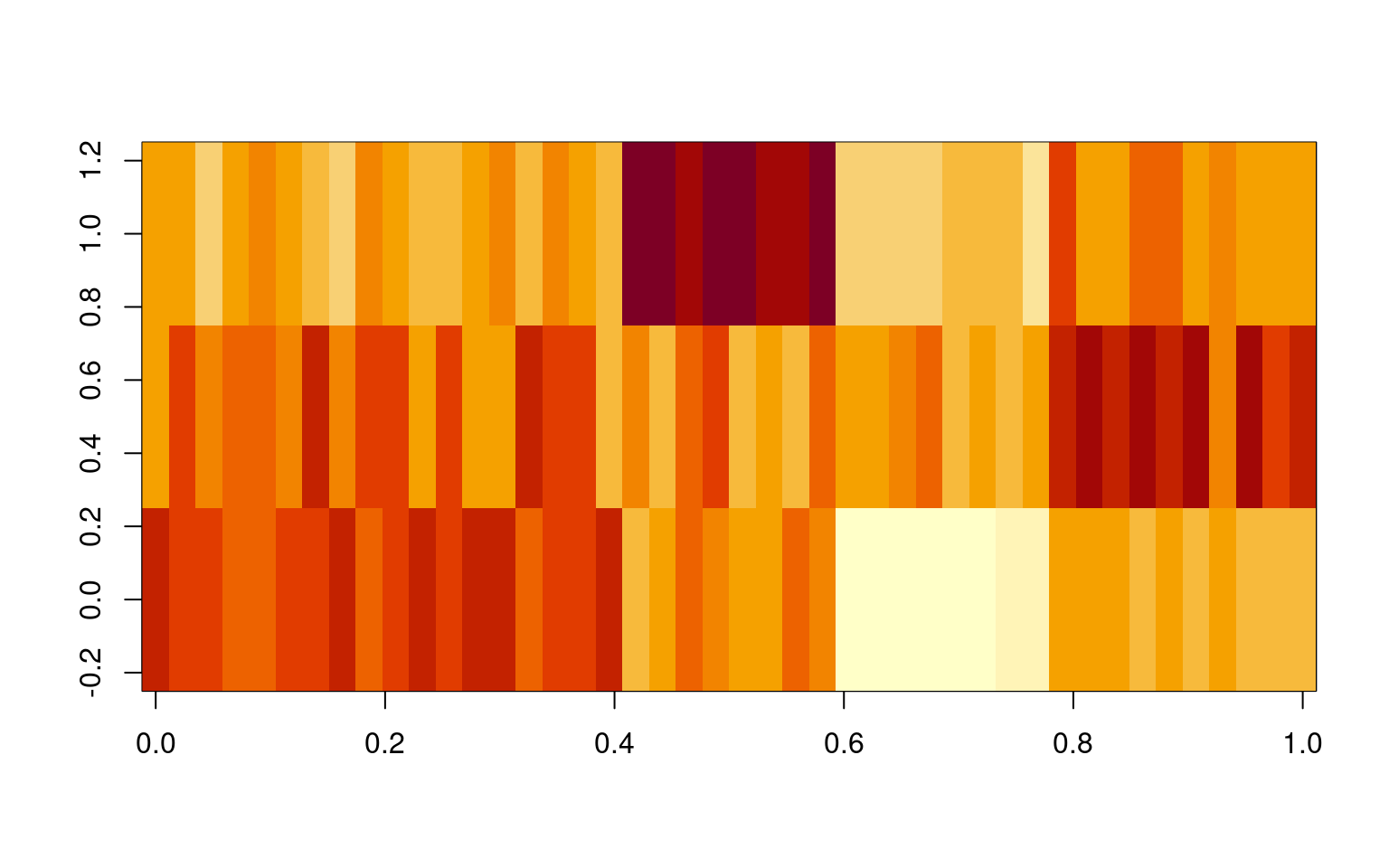## fairly similar: matplot(promax(loadings(f2))$loadings, type = "s", lty = 1, main = "promax rotation",
ylab = "Factor loading")matplot(oblimin(loadings(f2))$loadings, type = "s", lty = 1, main = "promax rotation", ylab = "Factor loading")matplot(simplimax(loadings(f2))$loadings, type = "s", lty = 1, main = "simplimax rotation",
ylab = "Factor loading")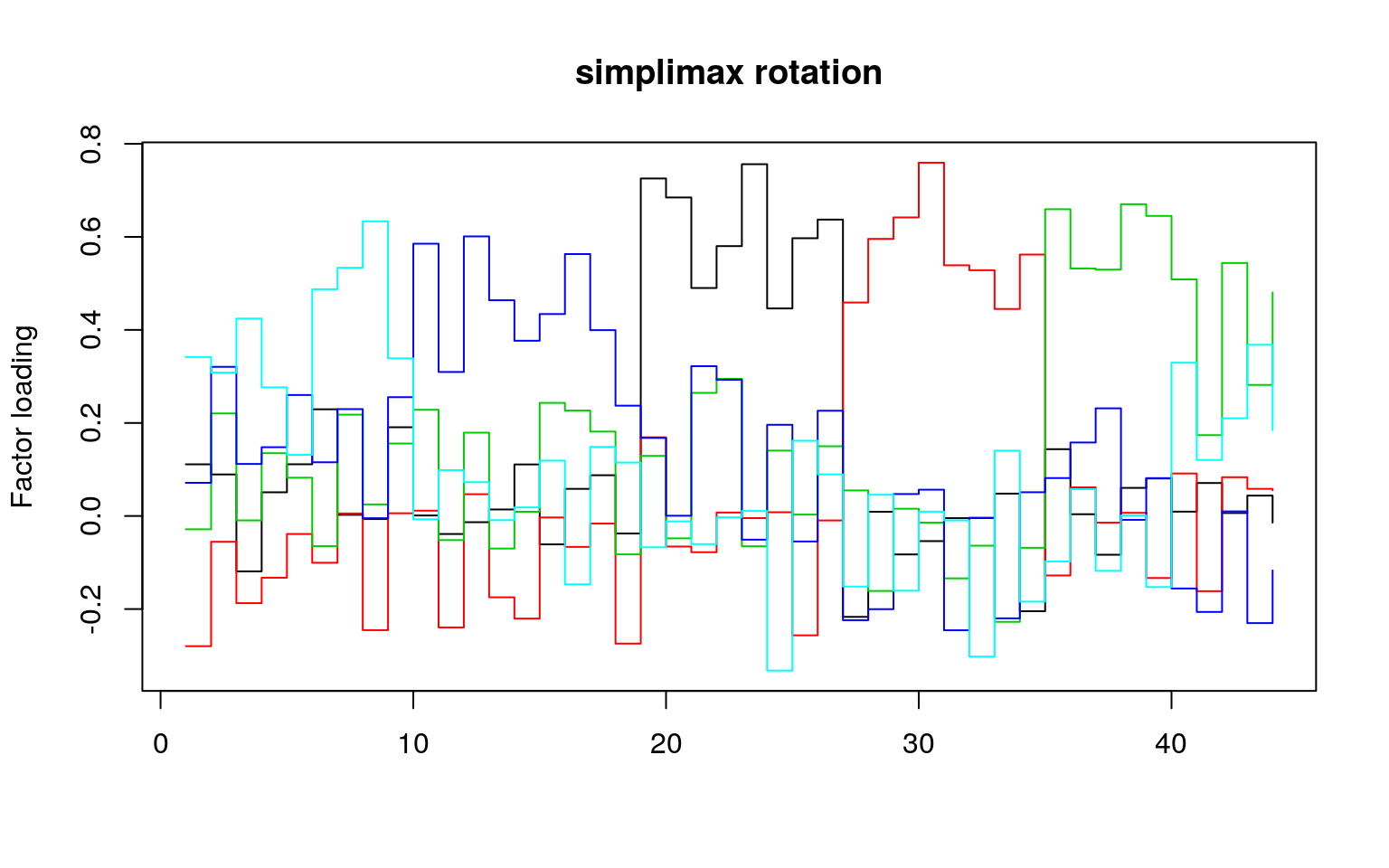matplot(oblimin(loadings(f2))$loadings, type = "s", lty = 1, main = "oblimin rotation", ylab = "Factor loading")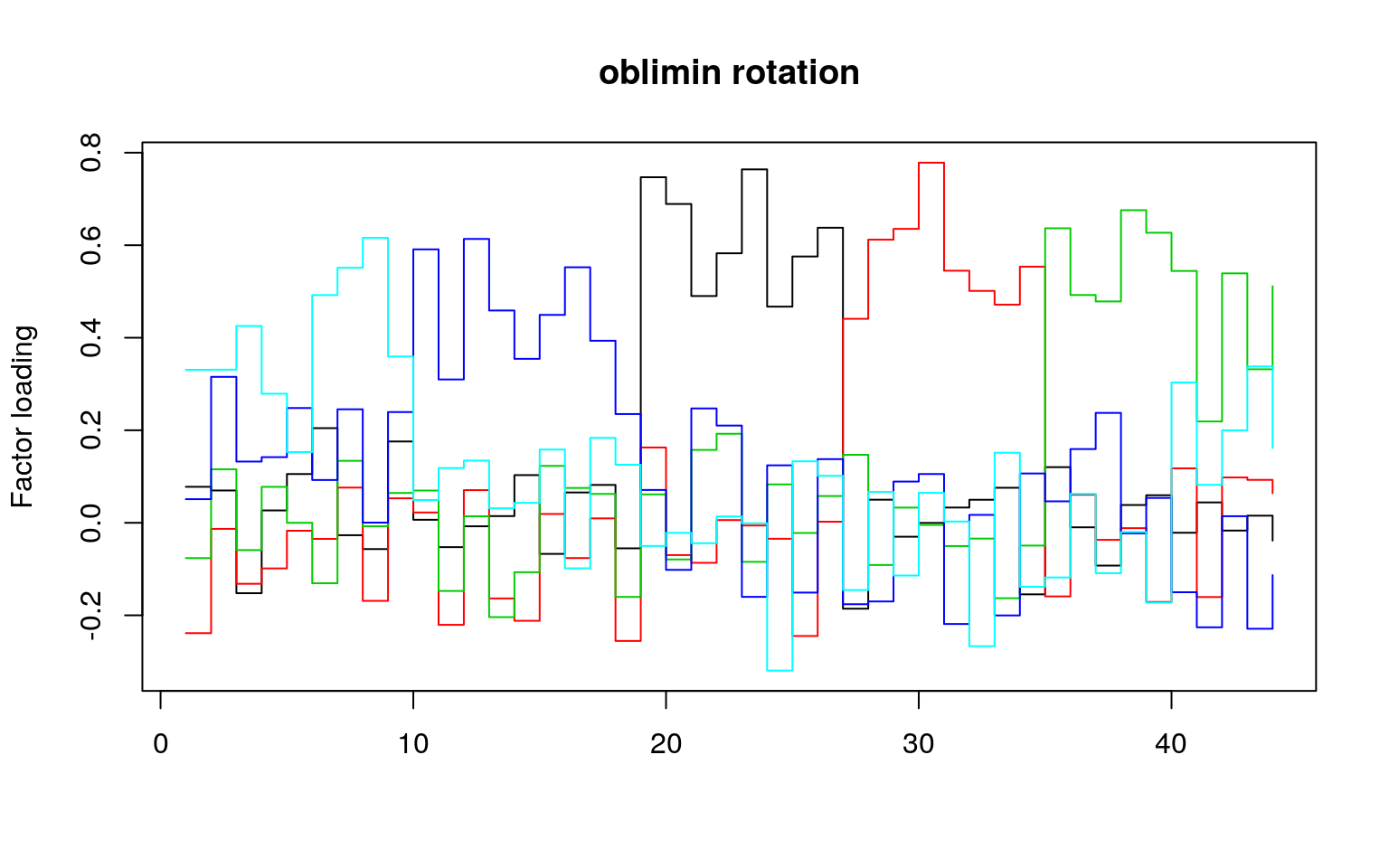matplot(varimin(loadings(f2))$loadings, type = "s", lty = 1, main = "varimin rotation",
ylab = "Factor loading")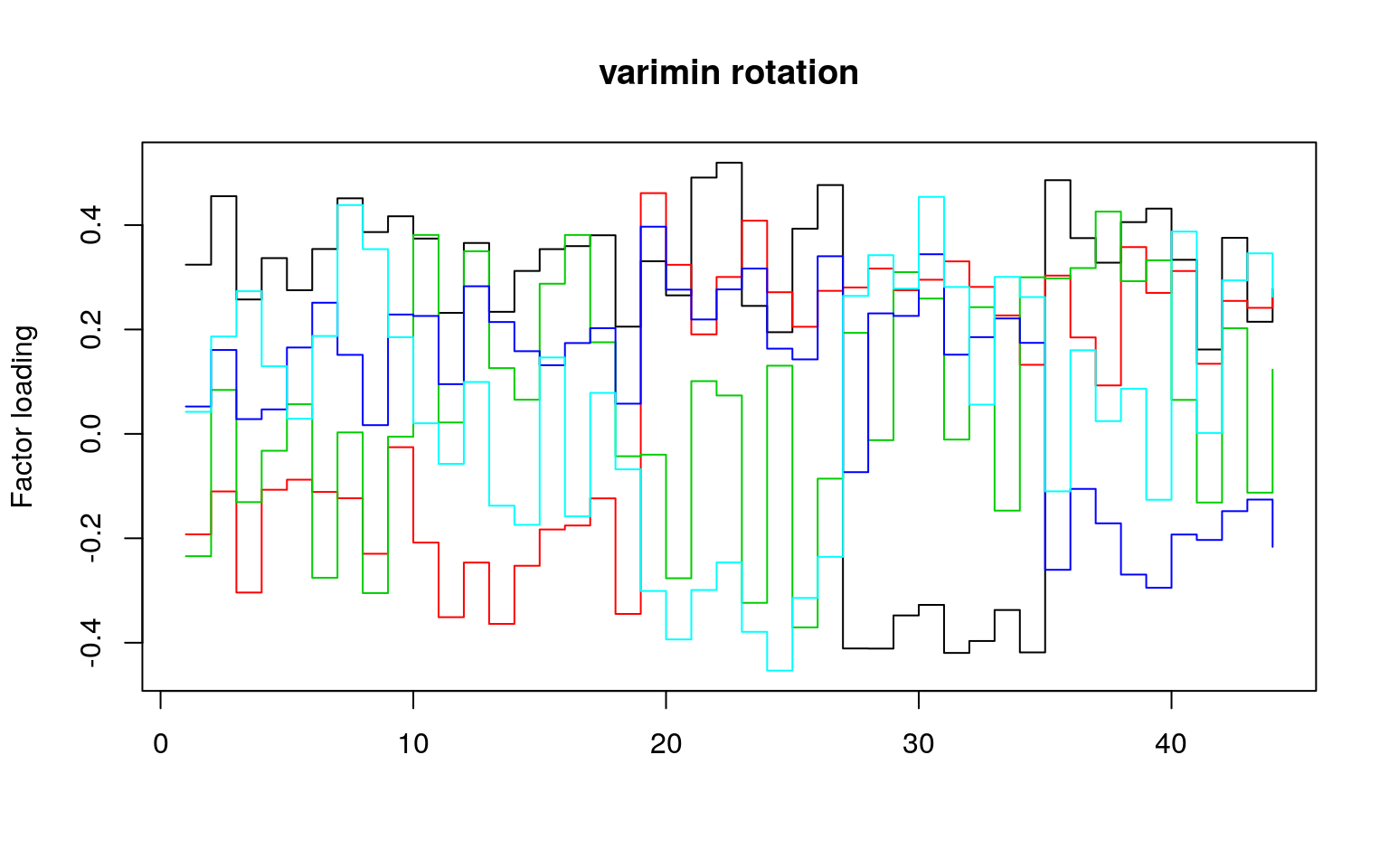## Oblique rotations

If you use an oblique rotation, that means your factors are allowed to be correlated. Here is the full output of an oblique rotation (the oblimin):

oblimin(loadings(f2))
Oblique rotation method Oblimin Quartimin converged.
ML2      ML3       ML4       ML1      ML5
A1   0.077792 -0.23858 -7.63e-02  0.051032  0.33072
A2   0.069772 -0.01312  1.16e-01  0.315380  0.33088
A3  -0.152205 -0.13186 -5.90e-02  0.132431  0.42540
A4   0.026625 -0.09872  7.76e-02  0.141818  0.27891
A5   0.105436 -0.01721 -2.08e-05  0.248122  0.15284
A6   0.204566 -0.03480 -1.31e-01  0.092521  0.49229
A7  -0.026877  0.07607  1.34e-01  0.245298  0.55135
A8  -0.056847 -0.16881 -7.57e-03  0.000358  0.61589
A9   0.175793  0.05282  6.45e-02  0.239280  0.35933
C10  0.006554  0.02231  6.97e-02  0.590989  0.04922
C11 -0.052645 -0.22064 -1.47e-01  0.309812  0.11829
C12 -0.007519  0.07060  1.41e-02  0.613554  0.13442
C13  0.014447 -0.16356 -2.04e-01  0.458906  0.03127
C14  0.102986 -0.21189 -1.07e-01  0.354427  0.04308
C15 -0.067266  0.01906  1.23e-01  0.449388  0.15831
[ reached getOption("max.print") -- omitted 29 rows ]

Rotating matrix:
[,1]    [,2]     [,3]    [,4]     [,5]
[1,]  1.0220  0.0854 -0.05952 -0.0134 -0.00594
[2,]  0.1084  1.0237 -0.00743  0.0509  0.01660
[3,] -0.1000 -0.0287  1.02863 -0.0821 -0.04556
[4,] -0.0488  0.1211 -0.14493  1.0445 -0.04486
[5,]  0.0114  0.0449 -0.02073 -0.0612  1.00868

Phi:
[,1]    [,2]    [,3]   [,4]    [,5]
[1,]  1.0000 -0.1931  0.1603  0.113  0.0184
[2,] -0.1931  1.0000 -0.0149 -0.179 -0.0729
[3,]  0.1603 -0.0149  1.0000  0.230  0.0818
[4,]  0.1135 -0.1794  0.2296  1.000  0.1240
[5,]  0.0184 -0.0729  0.0818  0.124  1.0000

Here, no factors are correlated more than around .2 (see the Phi matrix) which is a constraint in the oblique rotation. The oblique rotations/transformations will sacrifice orthogonality a little in order to maximize another property, usually something about the shared variance of the items that load highly on a factor.

## Choosing the right number of factors and evaluating goodness of fit

Like all of the methods we have used, it is tough to know how many factors there are. One approach is to use pca to identify how many factors to use (looking at the scree plot and eigen decomposition). Then do a factor analysis to pick a model with that many dimenions. You can also use the goodness-of-fit statistics reported in the model. Many times, the challenge is that in order to get a model whose goodness-of-fit is large enough to satisfy the basic advice, you need to add more factors than are really interpretable.

Several statistics are available for evaluating goodness of fit, and these can be used to assess the number of factors as well. Detailed tutorials are available at http://davidakenny.net/cm/fit.htm .

Many of the statistics are output from the factor analysis directly, but it may be convenient to use the VSS on the correlation matrix.

fac <- fa(bytype, nfactors = 5)
summary(fac)

Factor analysis with Call: fa(r = bytype, nfactors = 5)

Test of the hypothesis that 5 factors are sufficient.
The degrees of freedom for the model is 736  and the objective function was  3.2
The number of observations was  1017  with Chi Square =  3193.66  with prob <  4.9e-302

The root mean square of the residuals (RMSA) is  0.05
The df corrected root mean square of the residuals is  0.05

Tucker Lewis Index of factoring reliability =  0.762
RMSEA index =  0.058  and the 10 % confidence intervals are  0.055 0.059
BIC =  -1902.86
With factor correlations of
MR3   MR4  MR2   MR1   MR5
MR3  1.00 -0.19 0.16  0.13  0.02
MR4 -0.19  1.00 0.00 -0.20 -0.08
MR2  0.16  0.00 1.00  0.23  0.08
MR1  0.13 -0.20 0.23  1.00  0.14
MR5  0.02 -0.08 0.08  0.14  1.00
fac8 <- fa(bytype, nfactors = 8)
summary(fac8)

Factor analysis with Call: fa(r = bytype, nfactors = 8)

Test of the hypothesis that 8 factors are sufficient.
The degrees of freedom for the model is 622  and the objective function was  1.35
The number of observations was  1017  with Chi Square =  1342.04  with prob <  6.3e-55

The root mean square of the residuals (RMSA) is  0.02
The df corrected root mean square of the residuals is  0.03

Tucker Lewis Index of factoring reliability =  0.917
RMSEA index =  0.034  and the 10 % confidence intervals are  0.031 0.036
BIC =  -2965.07
With factor correlations of
MR3   MR4   MR2   MR5   MR6   MR8   MR7   MR1
MR3  1.00 -0.18  0.16 -0.03  0.12  0.01  0.06  0.13
MR4 -0.18  1.00 -0.09 -0.10 -0.30 -0.09 -0.05 -0.12
MR2  0.16 -0.09  1.00  0.01 -0.04  0.20  0.20  0.11
MR5 -0.03 -0.10  0.01  1.00  0.10 -0.01  0.17  0.06
MR6  0.12 -0.30 -0.04  0.10  1.00  0.18  0.03  0.02
MR8  0.01 -0.09  0.20 -0.01  0.18  1.00  0.22  0.28
MR7  0.06 -0.05  0.20  0.17  0.03  0.22  1.00  0.08
MR1  0.13 -0.12  0.11  0.06  0.02  0.28  0.08  1.00
fac10 <- fa(bytype, nfactors = 10)
fac10
Factor Analysis using method =  minres
Call: fa(r = bytype, nfactors = 10)
MR3   MR4   MR2   MR6   MR1   MR8   MR9   MR7  MR10   MR5   h2   u2
A1   0.04 -0.06  0.01  0.21  0.41 -0.10  0.02 -0.18  0.04  0.17 0.34 0.66
A2   0.08 -0.04  0.06  0.02  0.51  0.24  0.02  0.02 -0.07 -0.04 0.39 0.61
A3  -0.10  0.02 -0.03  0.13  0.28  0.17  0.04 -0.13 -0.12  0.37 0.37 0.63
A4  -0.06 -0.11  0.07 -0.07  0.61 -0.03 -0.03 -0.07  0.07 -0.02 0.40 0.60
A5  -0.04 -0.07  0.00  0.00  0.48 -0.01 -0.04  0.00  0.20 -0.14 0.30 0.70
com
A1  2.6
A2  1.6
A3  3.5
A4  1.2
A5  1.6
[ reached getOption("max.print") -- omitted 39 rows ]

MR3  MR4  MR2  MR6  MR1  MR8  MR9  MR7 MR10  MR5
SS loadings           2.95 2.83 2.43 2.11 2.02 1.83 1.66 1.49 1.39 1.19
Proportion Var        0.07 0.06 0.06 0.05 0.05 0.04 0.04 0.03 0.03 0.03
Cumulative Var        0.07 0.13 0.19 0.23 0.28 0.32 0.36 0.39 0.43 0.45
Proportion Explained  0.15 0.14 0.12 0.11 0.10 0.09 0.08 0.07 0.07 0.06
Cumulative Proportion 0.15 0.29 0.41 0.52 0.62 0.71 0.80 0.87 0.94 1.00

With factor correlations of
MR3   MR4   MR2   MR6   MR1   MR8   MR9   MR7  MR10   MR5
MR3   1.00 -0.15  0.18  0.10  0.03 -0.06  0.02  0.07  0.32  0.11
MR4  -0.15  1.00 -0.18 -0.27 -0.13 -0.04  0.11 -0.12 -0.10 -0.18
MR2   0.18 -0.18  1.00  0.00  0.08  0.21  0.38  0.09  0.17 -0.05
MR6   0.10 -0.27  0.00  1.00  0.11  0.15 -0.08  0.08  0.00  0.19
MR1   0.03 -0.13  0.08  0.11  1.00  0.21  0.18  0.16  0.17  0.29
MR8  -0.06 -0.04  0.21  0.15  0.21  1.00  0.10  0.21  0.22 -0.04
MR9   0.02  0.11  0.38 -0.08  0.18  0.10  1.00  0.24 -0.06  0.07
[ reached getOption("max.print") -- omitted 3 rows ]

Mean item complexity =  2.2
Test of the hypothesis that 10 factors are sufficient.

The degrees of freedom for the null model are  946  and the objective function was  14.28 with Chi Square of  14284.11
The degrees of freedom for the model are 551  and the objective function was  0.98

The root mean square of the residuals (RMSR) is  0.02
The df corrected root mean square of the residuals is  0.02

The harmonic number of observations is  1017 with the empirical chi square  602.99  with prob <  0.062
The total number of observations was  1017  with Likelihood Chi Square =  970.8  with prob <  1.3e-25

Tucker Lewis Index of factoring reliability =  0.946
RMSEA index =  0.028  and the 90 % confidence intervals are  0.025 0.03
BIC =  -2844.66
Fit based upon off diagonal values = 0.99
MR3  MR4  MR2  MR6  MR1
Correlation of (regression) scores with factors   0.93 0.92 0.91 0.88 0.87
Multiple R square of scores with factors          0.86 0.84 0.82 0.77 0.76
Minimum correlation of possible factor scores     0.73 0.69 0.64 0.55 0.52
MR8  MR9  MR7 MR10  MR5
Correlation of (regression) scores with factors   0.86 0.88 0.84 0.85 0.81
Multiple R square of scores with factors          0.75 0.77 0.71 0.71 0.66
Minimum correlation of possible factor scores     0.49 0.55 0.42 0.43 0.31
summary(fac10)

Factor analysis with Call: fa(r = bytype, nfactors = 10)

Test of the hypothesis that 10 factors are sufficient.
The degrees of freedom for the model is 551  and the objective function was  0.98
The number of observations was  1017  with Chi Square =  970.8  with prob <  1.3e-25

The root mean square of the residuals (RMSA) is  0.02
The df corrected root mean square of the residuals is  0.02

Tucker Lewis Index of factoring reliability =  0.946
RMSEA index =  0.028  and the 10 % confidence intervals are  0.025 0.03
BIC =  -2844.66
With factor correlations of
MR3   MR4   MR2   MR6   MR1   MR8   MR9   MR7  MR10   MR5
MR3   1.00 -0.15  0.18  0.10  0.03 -0.06  0.02  0.07  0.32  0.11
MR4  -0.15  1.00 -0.18 -0.27 -0.13 -0.04  0.11 -0.12 -0.10 -0.18
MR2   0.18 -0.18  1.00  0.00  0.08  0.21  0.38  0.09  0.17 -0.05
MR6   0.10 -0.27  0.00  1.00  0.11  0.15 -0.08  0.08  0.00  0.19
MR1   0.03 -0.13  0.08  0.11  1.00  0.21  0.18  0.16  0.17  0.29
MR8  -0.06 -0.04  0.21  0.15  0.21  1.00  0.10  0.21  0.22 -0.04
MR9   0.02  0.11  0.38 -0.08  0.18  0.10  1.00  0.24 -0.06  0.07
[ reached getOption("max.print") -- omitted 3 rows ]

One piece of advise is to examine the ‘Very Simple Structure’, and the psych package has an implementation of this. From the documentation for psych::VSS:

Techniques most commonly used include

1. Extracting factors until the chi square of the residual matrix is not significant.

2. Extracting factors until the change in chi square from factor n to factor n+1 is not significant.

3. Extracting factors until the eigen values of the real data are less than the corresponding eigen values of a random data set of the same size (parallel analysis) fa.parallel.

4. Plotting the magnitude of the successive eigen values and applying the scree test (a sudden drop in eigen values analogous to the change in slope seen when scrambling up the talus slope of a mountain and approaching the rock face.

5. Extracting principal components until the eigen value < 1.

6. Extracting factors as long as they are interpetable.

7. Using the Very Simple Structure Criterion (VSS).

8. Using Wayne Velicer’s Minimum Average Partial (MAP) criterion.

The VSS will display many of these criteria in a table. You can use any of these criteria, and we will look at many of them more specifically below. The output includes:

dof: degrees of freedom (if using FA)
chisq: chi square (from the factor analysis output (if using FA)
prob: probability of residual matrix > 0 (if using FA)
sqresid: squared residual correlations
RMSEA: the RMSEA for each number of factors
BIC: the BIC for each number of factors
eChiSq: the empirically found chi square
eRMS: Empirically found mean residual
eCRMS: Empirically found mean residual corrected for df
eBIC: The empirically found BIC based upon the eChiSq
fit: factor fit of the complete model
cfit.1: VSS fit of complexity 1
cfit.2: VSS fit of complexity 2
cfit.8: VSS fit of complexity 8
cresidiual.1: sum squared residual correlations for complexity 1
: sum squared residual correlations for complexity 2 ..8

Running VSS requires giving it the raw correlation matrix:

ff <- cor(bytype, use = "pairwise.complete")
VSS(ff, n = 10)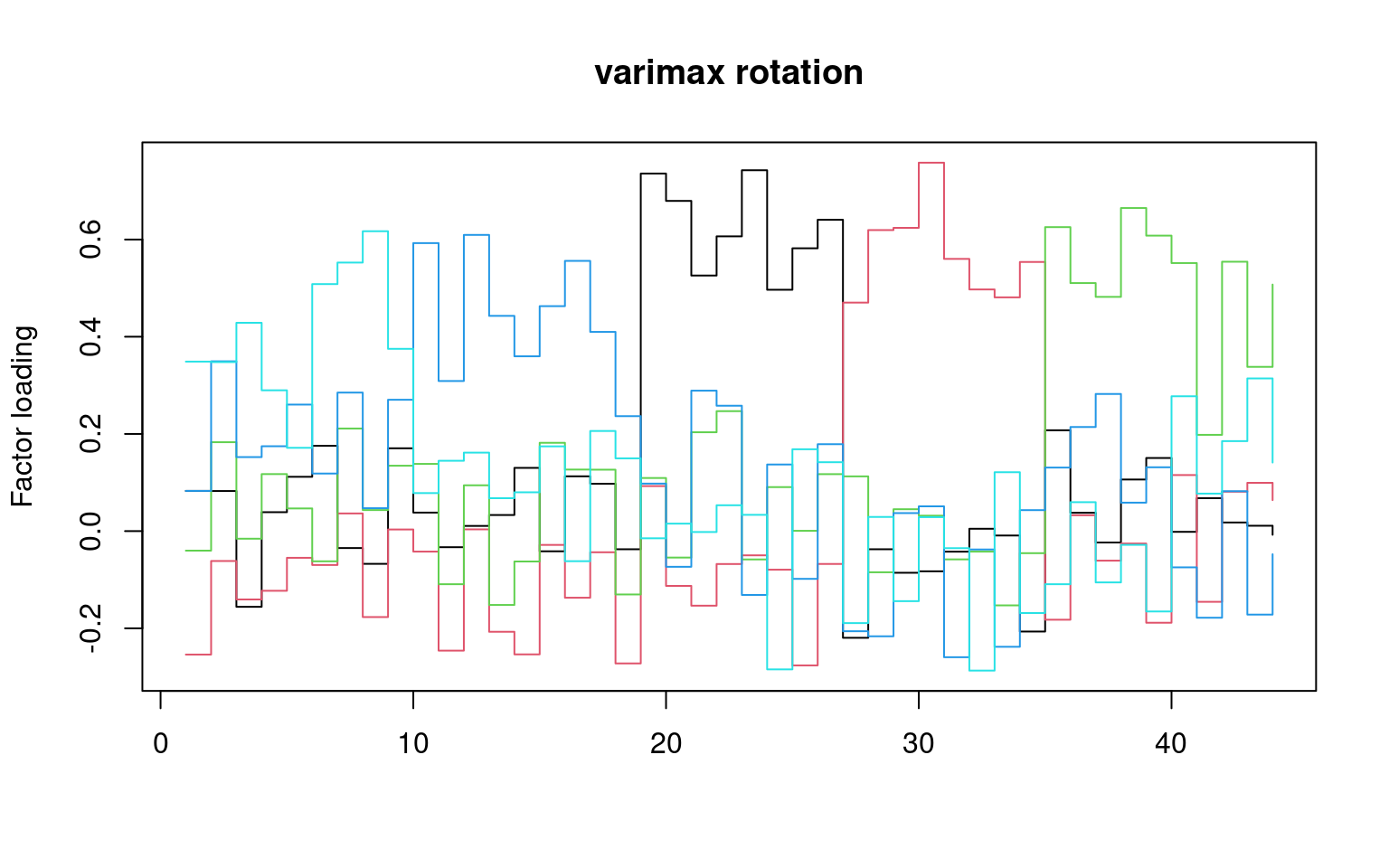Very Simple Structure
Call: vss(x = x, n = n, rotate = rotate, diagonal = diagonal, fm = fm,
n.obs = n.obs, plot = plot, title = title, use = use, cor = cor)
VSS complexity 1 achieves a maximimum of 0.52  with  6  factors
VSS complexity 2 achieves a maximimum of 0.67  with  5  factors

The Velicer MAP achieves a minimum of 0.01  with  7  factors
BIC achieves a minimum of  -2977.51  with  8  factors
Sample Size adjusted BIC achieves a minimum of  -1101.97  with  10  factors

Statistics by number of factors
vss1 vss2    map dof chisq     prob sqresid  fit RMSEA   BIC SABIC
1  0.42 0.00 0.0193 902  9845  0.0e+00      59 0.42 0.101  3614  6479
2  0.43 0.55 0.0162 859  7801  0.0e+00      46 0.55 0.091  1868  4596
3  0.49 0.64 0.0127 817  5617  0.0e+00      34 0.67 0.077   -26  2568
4  0.51 0.67 0.0106 776  4260  0.0e+00      28 0.72 0.068 -1101  1364
complex eChisq  SRMR eCRMS  eBIC
1      1.0  25559 0.116 0.119 19328
2      1.4  17254 0.095 0.100 11320
3      1.5   9725 0.072 0.077  4082
4      1.6   6604 0.059 0.065  1244
[ reached getOption("max.print") -- omitted 6 rows ]
VSS.scree(ff)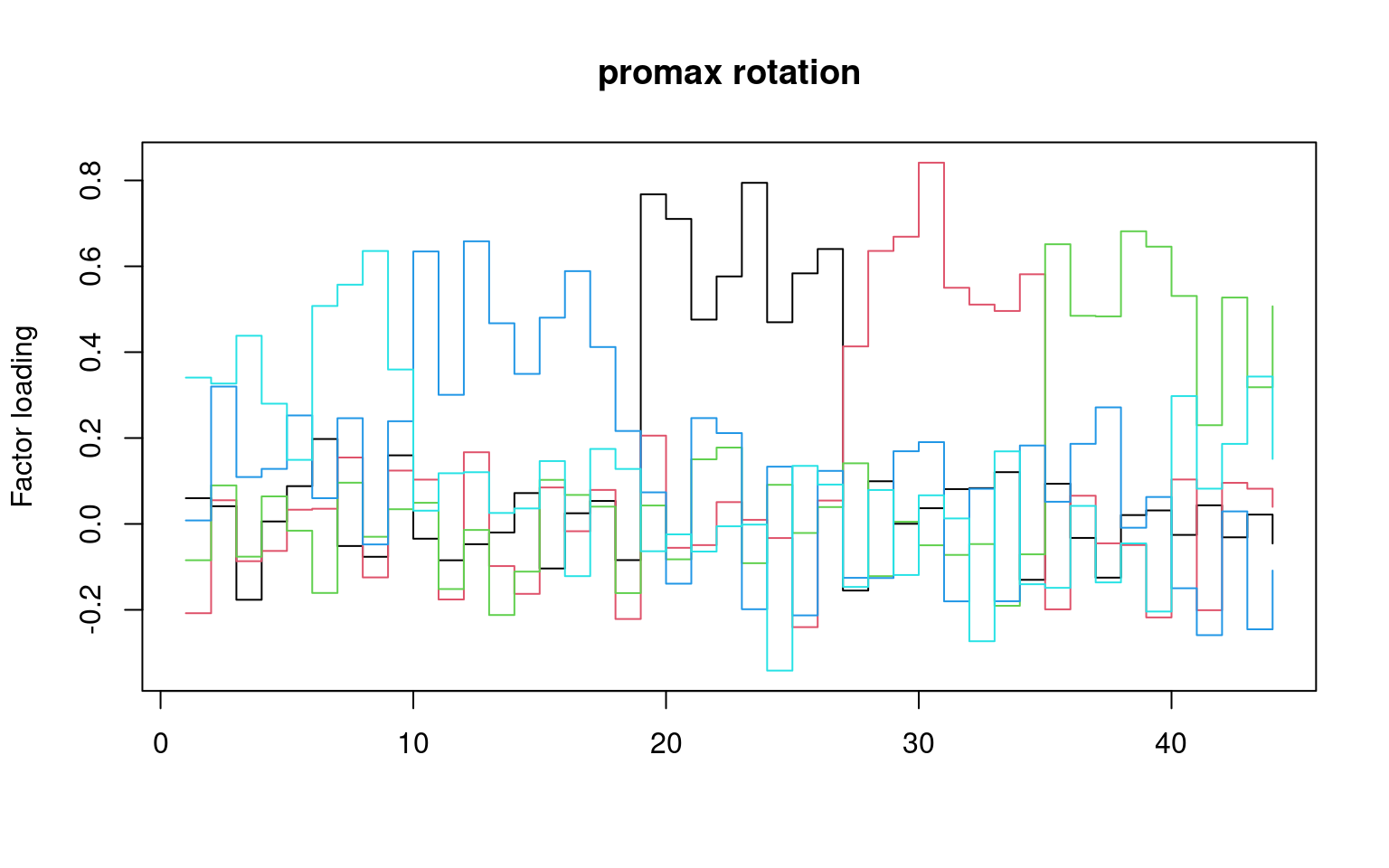## Summary of goodness-of-fit statistics.

### VSS

VSS scores tend to peak at a number of factors that is ‘most interpretable’. They compare the fit of the simplified model to the original correlations

### RMSEA

RMSEA score is a measure of room emas square error residuals. It says how far off you are, on average, when you compare the predicted correlation matrix and the actual one. Here, for 5 factors, it is .058, which is reasonably ‘good’, but not great (a RMSEA of .01 would be great). For 10 factors, it gets down to .028–but at what cost?

A RMSEA of .05 means that when you try to estimate the correlation matrix, each error is off by about .05.

### TLI

TLI is based on comparing expected versus observed chi-squared tests, penalizing by the size of the model. Here, it is .764 for 5 groups and .95 for 10 groups. A value of .9 is considered marginal, .95+ is good. So, 5 groups would be considered inadequate.

### BIC and AIC

BIC is a relative measure, which combines goodness of fit in its likelihood with model complexity. Smaller or more negative scores are better. Here, BIC for 5-factor is -1919, and for 10-factor it is -2854.69. The BIC, which is very reluctant to add additional parameters, thinks 10-factors is better than 5.

### MAP: Velicer’s Minimal Average Partial

This will tend to minimize for the ‘best’ model.

### Interpretability

More often than not, researchers rely on notions of interpretability to guide exploratory factor analysis. The statistics may tell you a ten-factor solution is needed, but you may only be able to interpret three. Ultimately, the goal of FA is usually scientific inference, and if the factor is not helping you understand anything, it might not be helpful to use.

## Examining and displaying the models

It is common to display the results in several ways that show the factor structure. if you use the plot method, it will show you the points along each pair of dimensions, with point color-coded by whether they are high or low on each dimension.

fa.diagram(fac)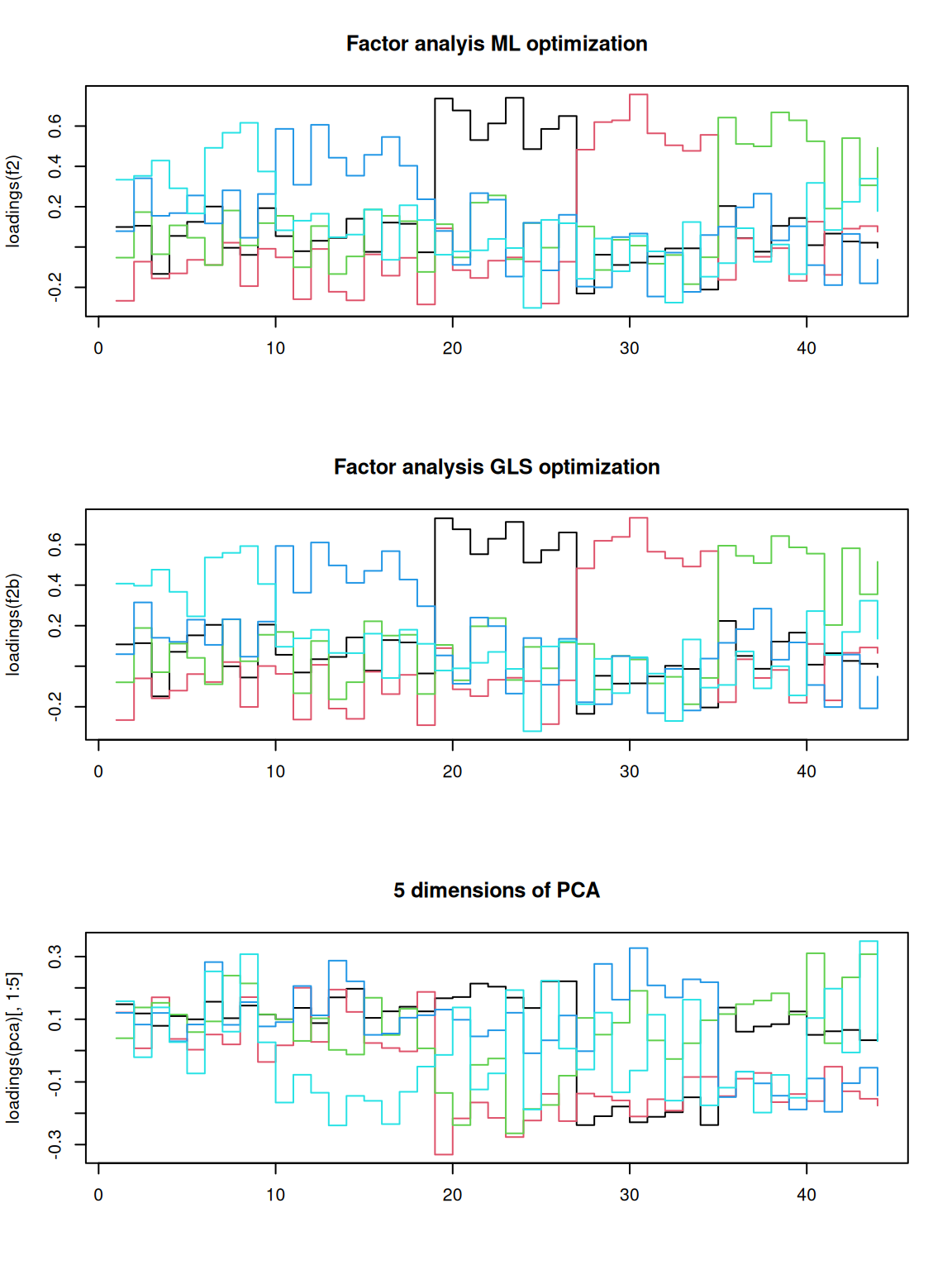fa.diagram(fac10)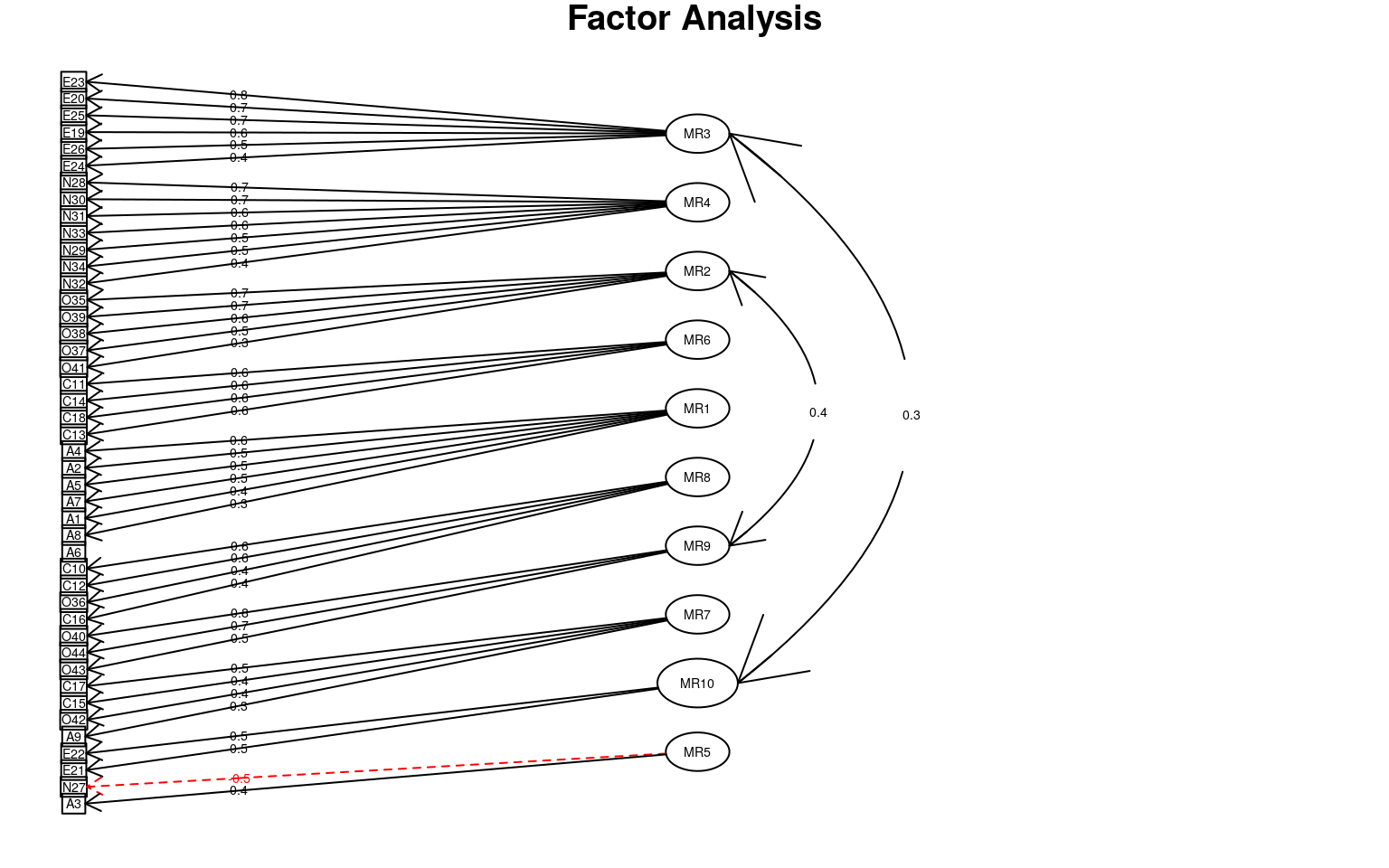fa.diagram(fac8)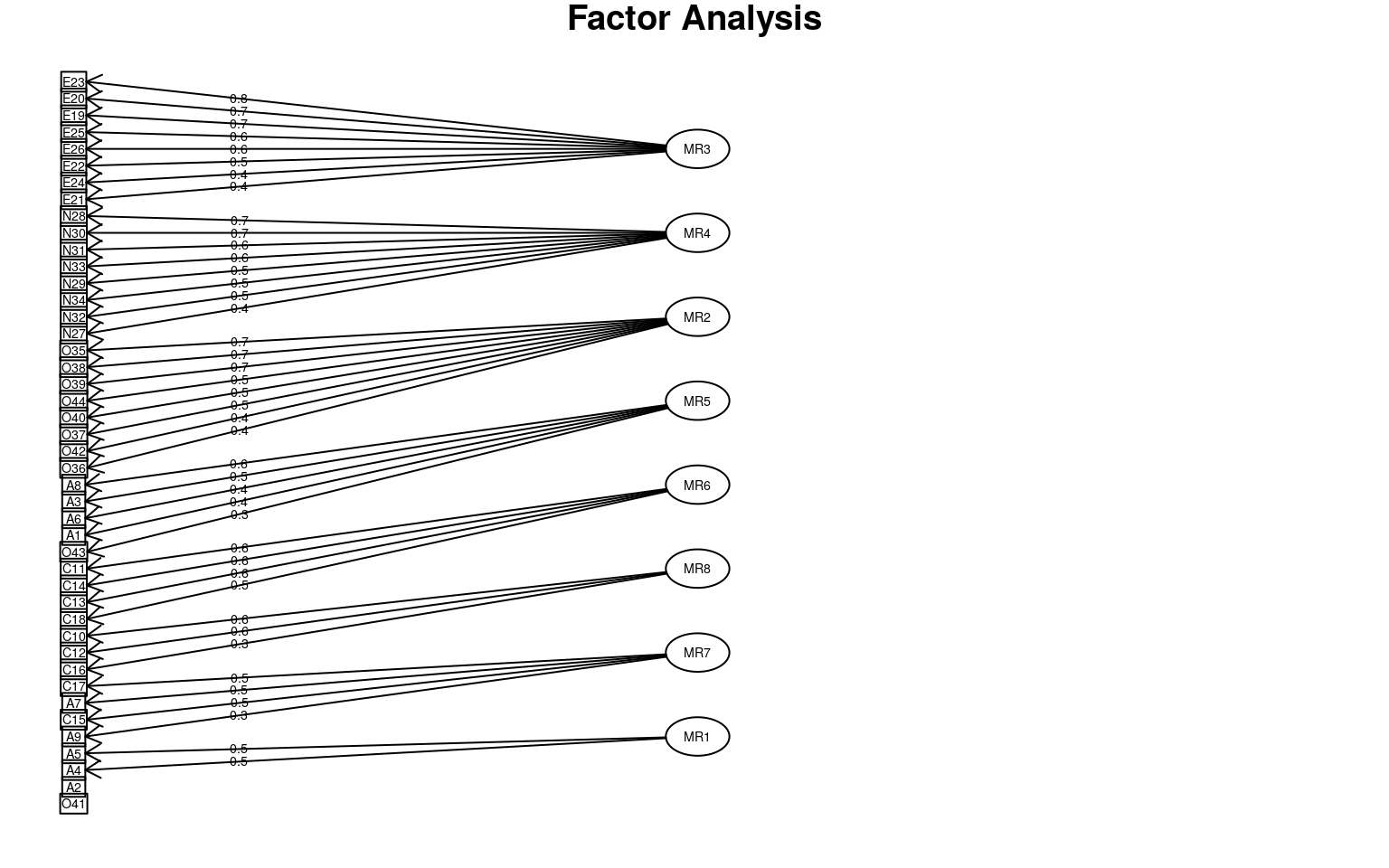## Plotting the factors:
fac4b <- fa(bytype, nfactors = 4)
plot(fac4b)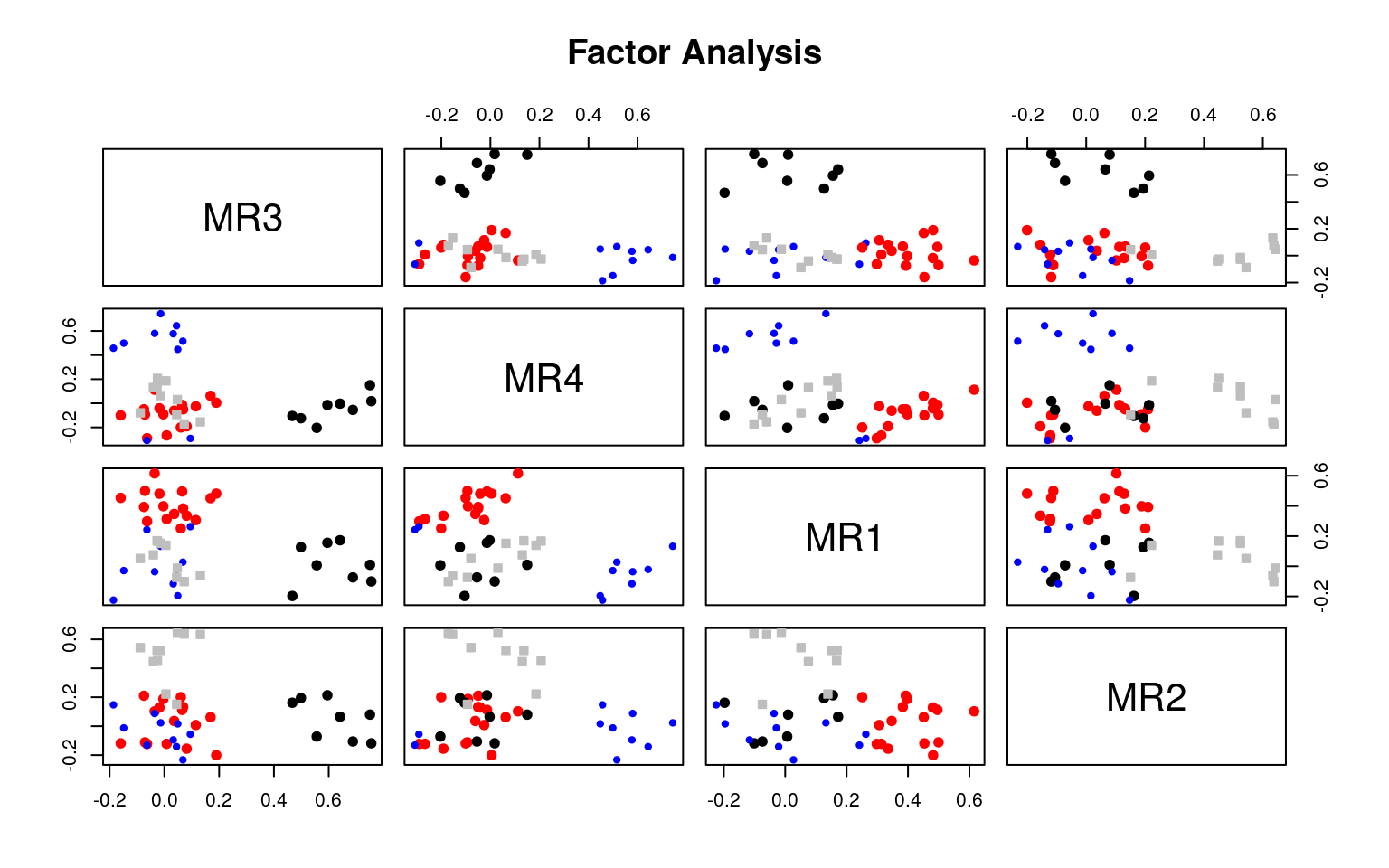fa.diagram(fac4b)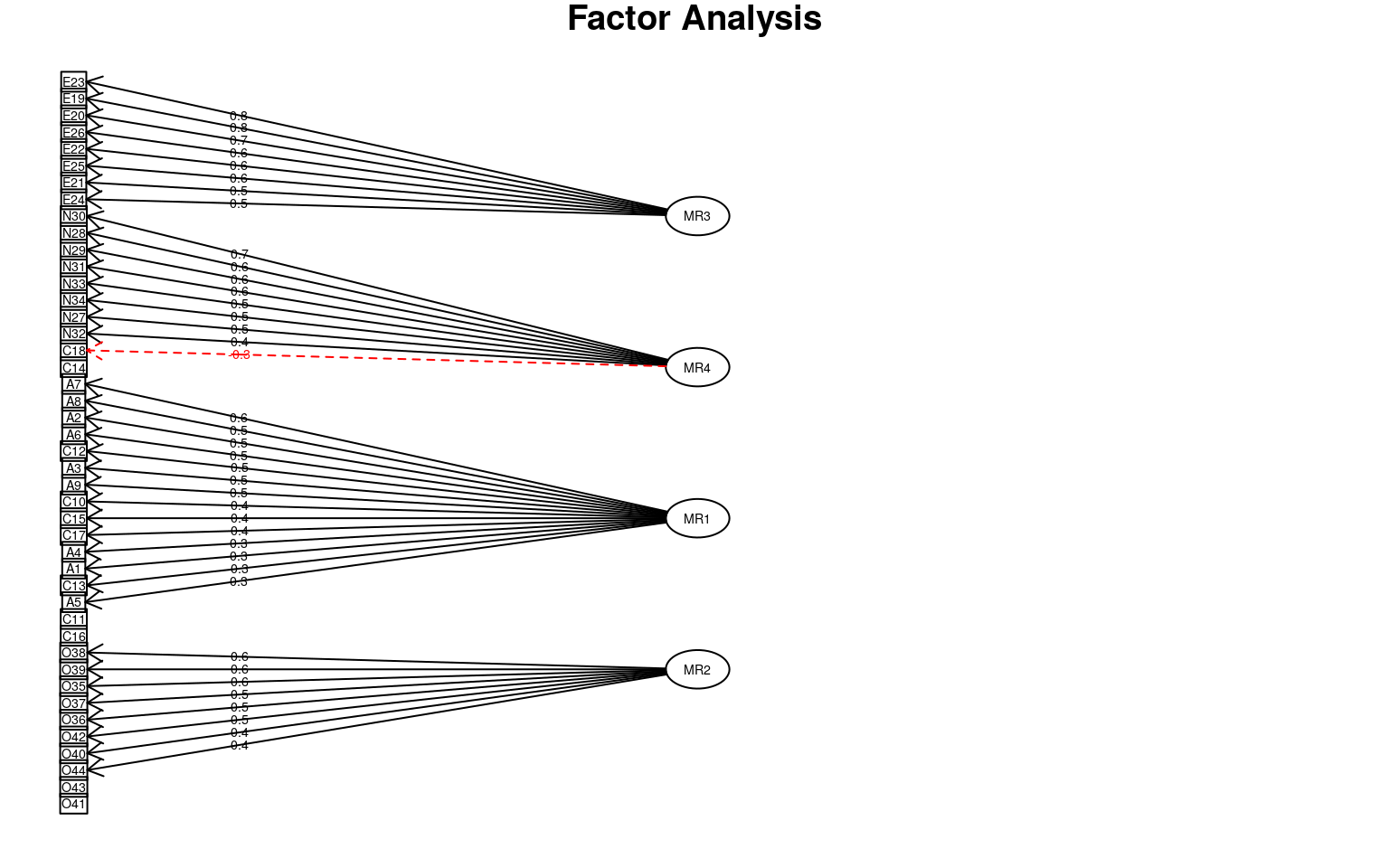Use the michigan house votes to compute a factor analysis. If necessary, determine what to do for ‘imupute’, figure out how many factors to use, explore several rotations, and try to identify an interpretable result.

# Assessing factors of political issues

First we can look at a PCA to get an idea of the number of factors that might exist in the data.

library(factoextra)
rownames(votes) <- paste(house\$Party, 1:nrow(house), sep = "-")
pca <- princomp(~., data = votes, scores = T)  #this handles NA values
fviz_screeplot(pca)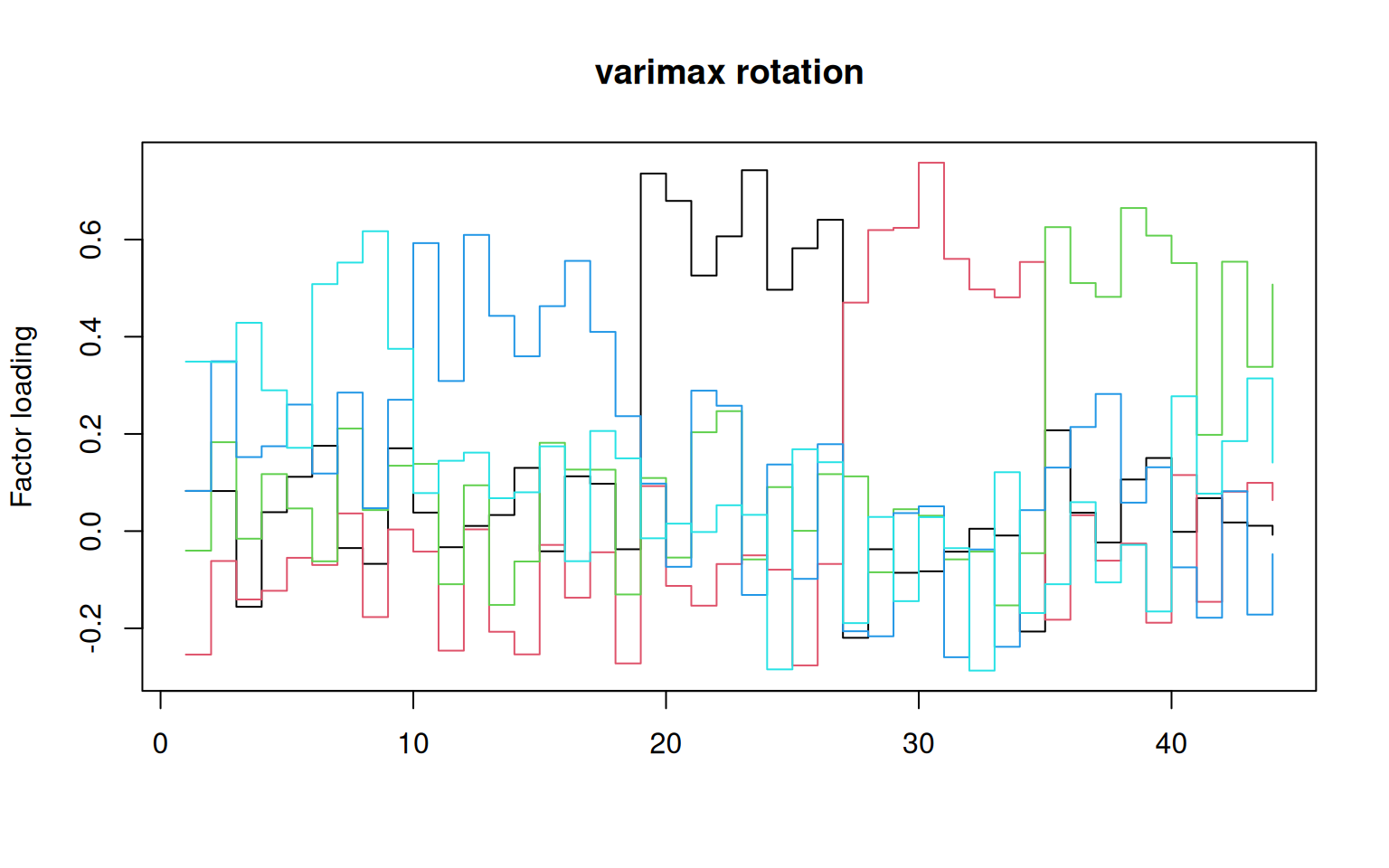fviz_pca_var(pca)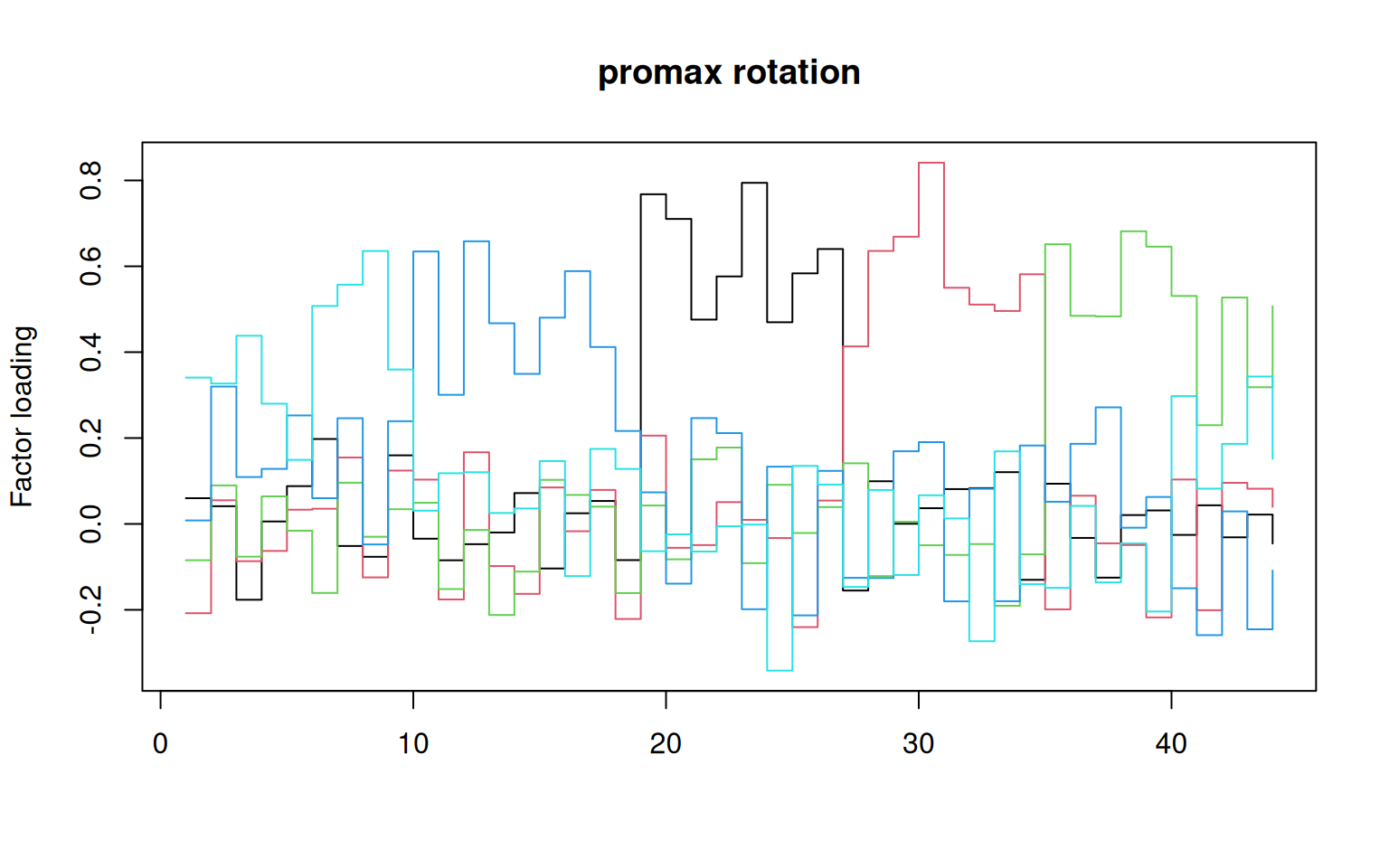fviz_pca(pca, col.ind = "cos2")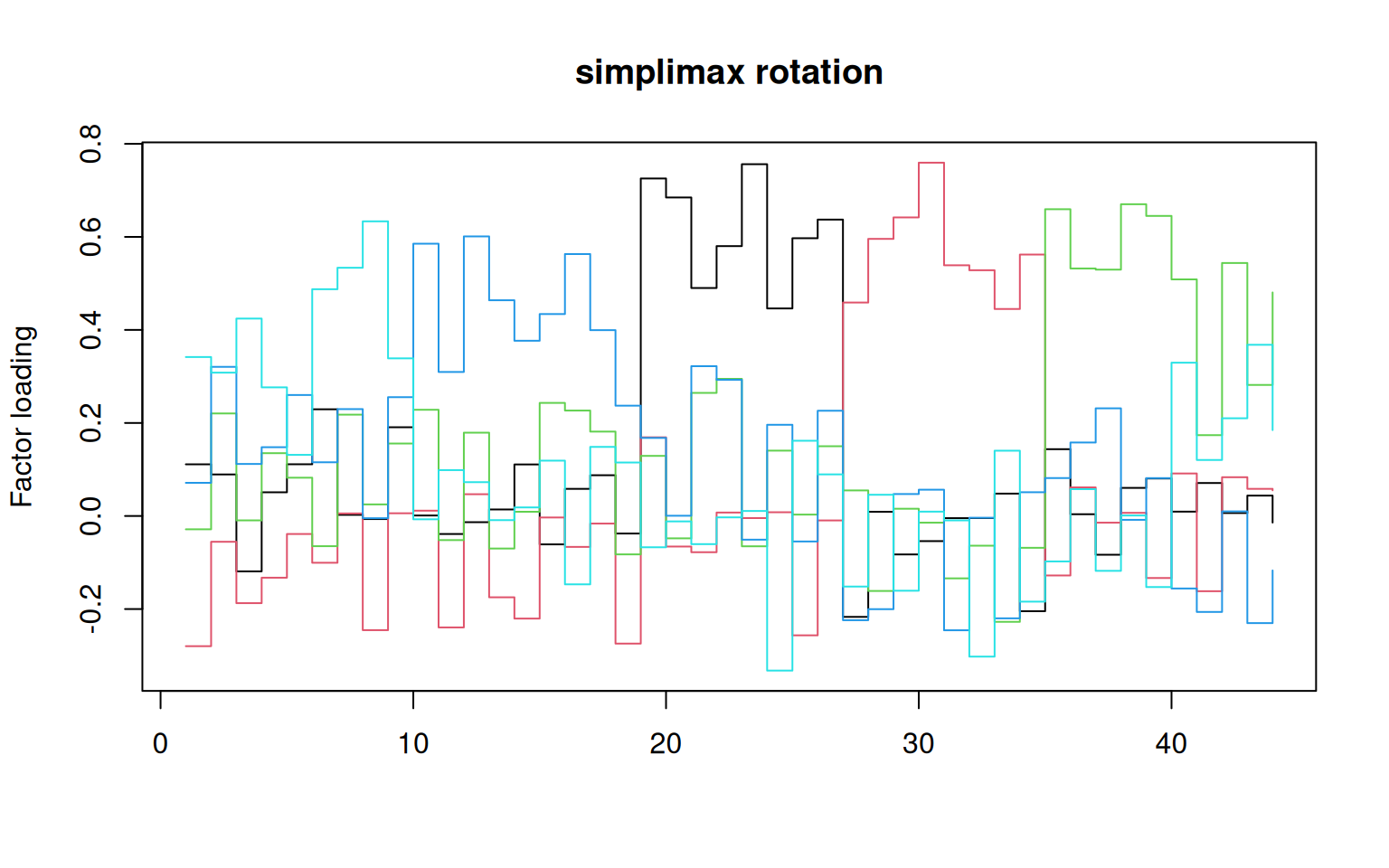print(pca)
Call:
princomp(formula = ~., data = votes, scores = T)

Standard deviations:
Comp.1     Comp.2     Comp.3     Comp.4     Comp.5     Comp.6
2.27298933 0.84883004 0.61473597 0.52132188 0.48197986 0.45073460
Comp.7     Comp.8     Comp.9    Comp.10    Comp.11    Comp.12
0.43112166 0.42431267 0.41106396 0.39977644 0.37296363 0.36746855
Comp.13    Comp.14    Comp.15    Comp.16    Comp.17    Comp.18
0.36208835 0.35478497 0.34330296 0.33065363 0.32432039 0.31664412
Comp.19    Comp.20    Comp.21    Comp.22    Comp.23    Comp.24
0.30303049 0.29492320 0.28551756 0.27993476 0.26451712 0.25354569
Comp.25    Comp.26    Comp.27    Comp.28    Comp.29    Comp.30
0.24797614 0.24107537 0.23097064 0.22455012 0.22218673 0.20988305
Comp.31    Comp.32    Comp.33    Comp.34    Comp.35    Comp.36
0.20171585 0.18798005 0.18474017 0.18368082 0.17029524 0.15998505
Comp.37    Comp.38    Comp.39    Comp.40    Comp.41    Comp.42
0.15194895 0.14373720 0.13993320 0.13420017 0.12630521 0.11776073
Comp.43    Comp.44    Comp.45    Comp.46    Comp.47    Comp.48
0.11428991 0.10488459 0.10316501 0.09802085 0.08799070 0.07692602
Comp.49    Comp.50    Comp.51    Comp.52    Comp.53    Comp.54
0.07392887 0.06341403 0.05926516 0.05214332 0.04332123 0.03207150

54  variables and  103 observations.
(loadings(pca))

Comp.1 Comp.2 Comp.3 Comp.4 Comp.5 Comp.6 Comp.7 Comp.8 Comp.9 Comp.10
Q1                        0.549  0.182  0.286         0.280  0.343  0.208
Comp.11 Comp.12 Comp.13 Comp.14 Comp.15 Comp.16 Comp.17 Comp.18
Q1   0.139   0.149           0.124   0.217   0.193
Comp.19 Comp.20 Comp.21 Comp.22 Comp.23 Comp.24 Comp.25 Comp.26
Q1           0.180   0.225                   0.112
Comp.27 Comp.28 Comp.29 Comp.30 Comp.31 Comp.32 Comp.33 Comp.34
Q1   0.122                   0.112
Comp.35 Comp.36 Comp.37 Comp.38 Comp.39 Comp.40 Comp.41 Comp.42
Q1
Comp.43 Comp.44 Comp.45 Comp.46 Comp.47 Comp.48 Comp.49 Comp.50
Q1
Comp.51 Comp.52 Comp.53 Comp.54
Q1
[ reached getOption("max.print") -- omitted 53 rows ]

Comp.1 Comp.2 Comp.3 Comp.4 Comp.5 Comp.6 Comp.7 Comp.8
Comp.9 Comp.10 Comp.11 Comp.12 Comp.13 Comp.14 Comp.15
Comp.16 Comp.17 Comp.18 Comp.19 Comp.20 Comp.21 Comp.22
Comp.23 Comp.24 Comp.25 Comp.26 Comp.27 Comp.28 Comp.29
Comp.30 Comp.31 Comp.32 Comp.33 Comp.34 Comp.35 Comp.36
Comp.37 Comp.38 Comp.39 Comp.40 Comp.41 Comp.42 Comp.43
Comp.44 Comp.45 Comp.46 Comp.47 Comp.48 Comp.49 Comp.50
Comp.51 Comp.52 Comp.53 Comp.54
[ reached getOption("max.print") -- omitted 2 rows ]

Questions:

• How many factors seem reasonable?
• Looking at those factors, Can you make any interpretations?

## try this with psych::principal, which will produce many of the same results as psych::fa

p2 <- psych::principal(votes, nfactors = 5)
p2
Principal Components Analysis
Call: psych::principal(r = votes, nfactors = 5)
RC1   RC2   RC3   RC5   RC4   h2    u2 com
Q1   0.19  0.05 -0.01 -0.15  0.66 0.50 0.501 1.3
Q2   0.68 -0.01  0.29  0.11  0.09 0.57 0.435 1.4
Q3   0.61 -0.11  0.27  0.15  0.13 0.50 0.498 1.7
Q4   0.12  0.66 -0.09  0.09  0.29 0.55 0.449 1.5
Q5  -0.20 -0.58  0.26 -0.02 -0.10 0.46 0.541 1.7
Q6   0.37  0.10  0.62  0.06 -0.05 0.54 0.464 1.7
Q7   0.95  0.07  0.08  0.04  0.01 0.91 0.091 1.0
Q8   0.78  0.12  0.25 -0.07  0.13 0.70 0.295 1.3
Q9   0.12  0.71  0.00  0.13 -0.11 0.55 0.455 1.2
[ reached getOption("max.print") -- omitted 45 rows ]

RC1  RC2  RC3  RC5  RC4
Proportion Var         0.39 0.07 0.07 0.05 0.04
Cumulative Var         0.39 0.46 0.54 0.59 0.62
Proportion Explained   0.62 0.12 0.12 0.08 0.06
Cumulative Proportion  0.62 0.74 0.86 0.94 1.00

Mean item complexity =  1.6
Test of the hypothesis that 5 components are sufficient.

The root mean square of the residuals (RMSR) is  0.05
with the empirical chi square  658.97  with prob <  1

Fit based upon off diagonal values = 0.99
plot(p2)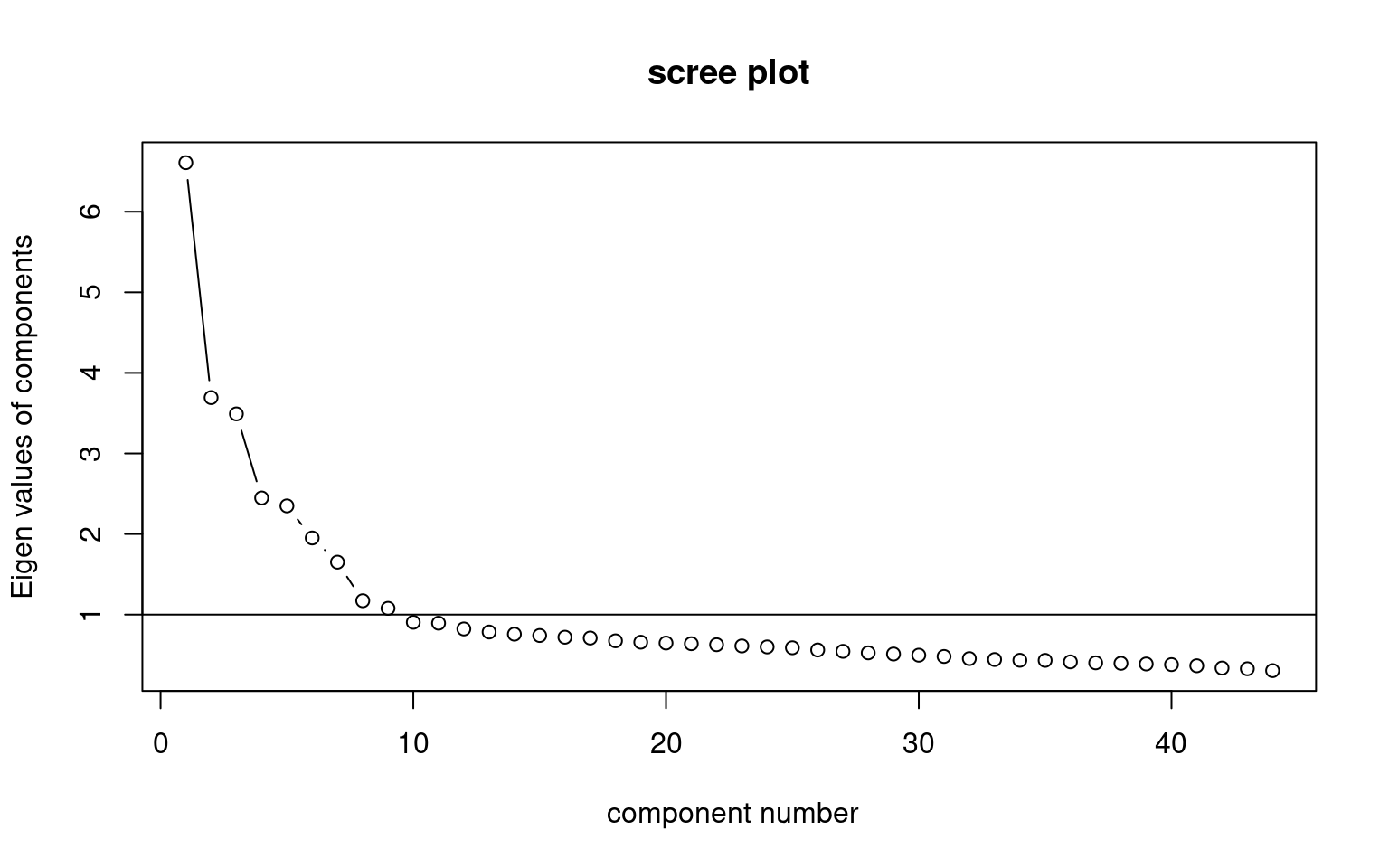## Factor analysis:

Perform a factor analysis with psych::fa. Determine how many dimensions are necessary, and justify model with goodness of fit parameters. - examine both orthogonal and non-orthogonal rotations - Create a plot showing the factor structure - examine models of different sizes to identify a good solution given the data set. - For the non-orthogonal rotation, examine correlations between factors.

Baseline solution:

efa5 <- psych::fa(r = votes, nfactors = 5)

2019-04-11